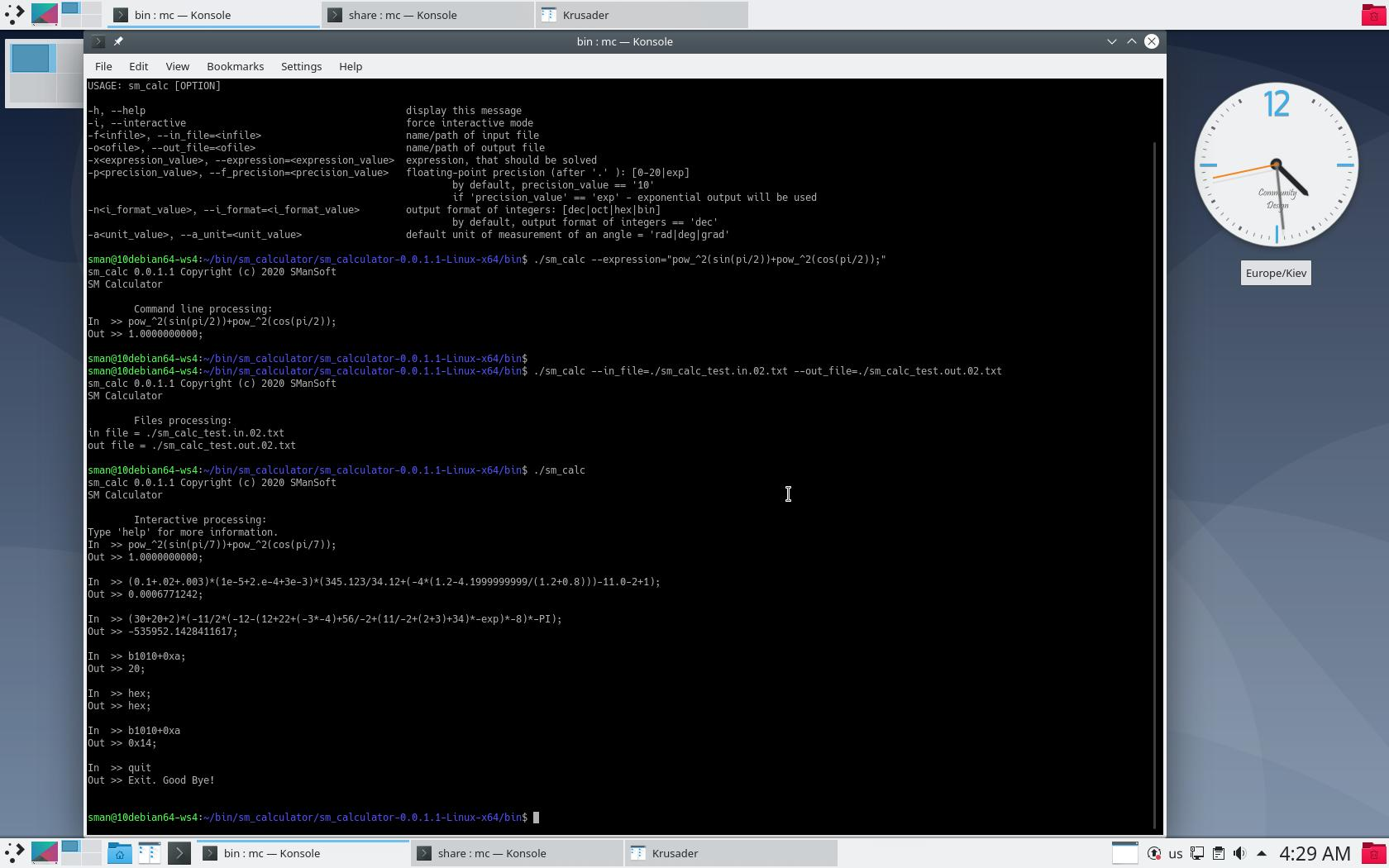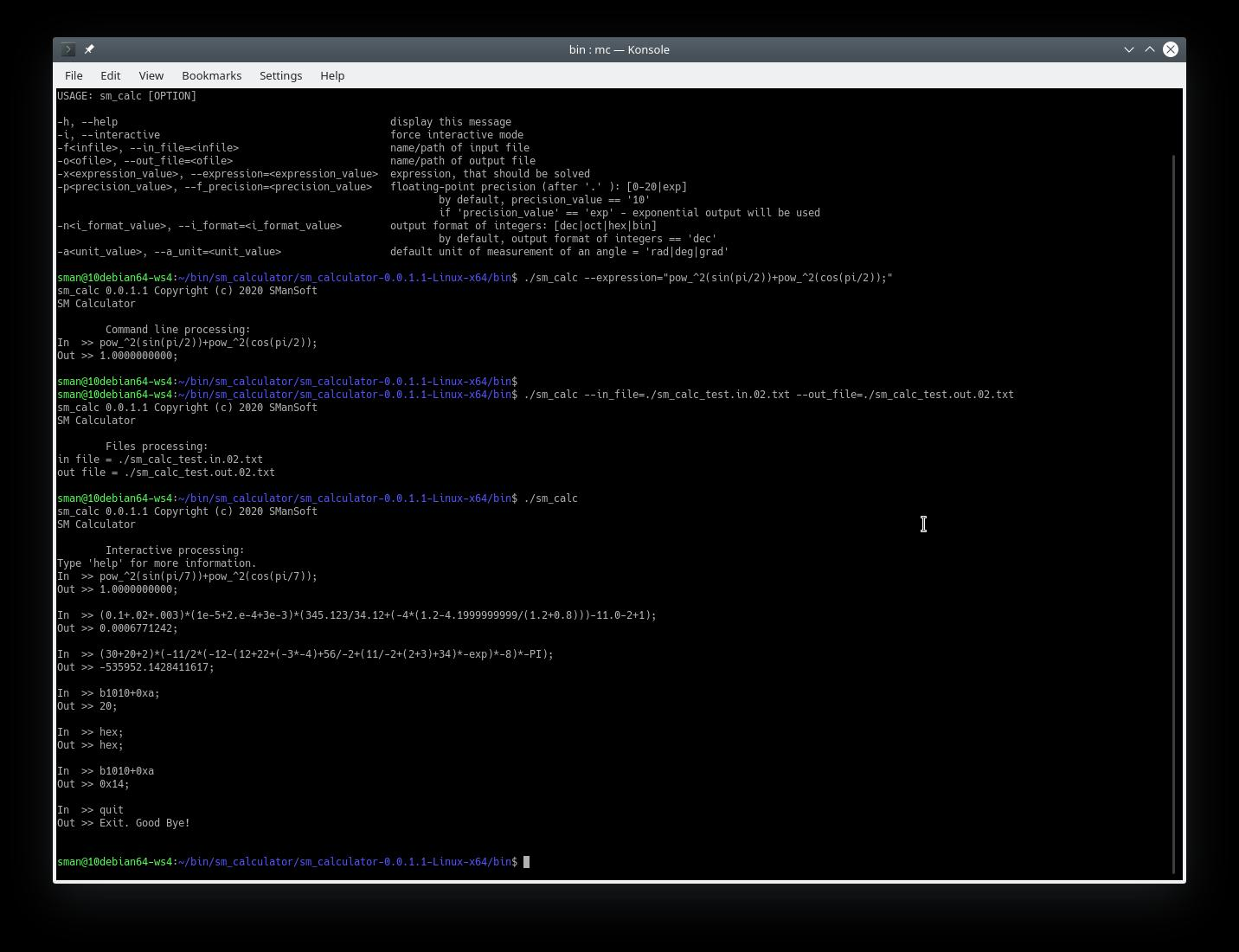Open-source Android VPN Stack Suite 0.0.1.1

Open-source Android VPN Stack Suite 0.0.1.1 has been released.

Supported platforms:

• Android (sdk >= 26)
• Examples (demo files, result files, log files), screenshots:
Examples

—————-

GPG Public Key (SManSoft ECDSA Key) <info@smansoft.com> has been published here: http://smansoft.com/gpg/smansoft.pub.asc.
Android VPN Stack Suite – 0.0.1.1 contains suite of [some_install_file].asc files.
gpg --import ./smansoft.pub.asc
for importing of key and
gpg --verify ./[some_install_file].asc ./[some_install_file]
or
gpg --verify ./[some_install_file].asc
for verification of files, published on this site.
Public GPG ECDSA Key can be imported, using
gpg --keyserver hkp://pgp.mit.edu --recv-keys A408B0FCFD774649
too.

Features of the Android VPN Stack Suite:

• Simple TCP/UDP socket server (with multi-thread support) (Linux platform), that implements open simple communication protocol (application level):
• TCP:
• The Client connects to the TCP Server;
• The Client sends “socket_client: Hello” to the Server;
• The Server reads message from the Client;
• The Server sends “socket_server: Hello” to the Client;
• The Client reads message from the Server;
• The Client sends some text message (size 1024 with ‘\0’ symbol)  to the Server;
• The Server reads text message from the Client and generates inverted message (src: “1234567890” -> inverted: “0987654321”);
• The Server sends inverted message to the Client;
• The Client reads message from the Server;
• The Client send read message to the Server;
• The Server reads text message from the Client and generates inverted message (src: “0987654321” -> inverted: “1234567890”);
• The Server sends inverted message to the Client;
• The Client reads message from the Server;
• Last received message by the Client should be similar to the first message sent by Client to the Server;
• UDP:
• The Client connects to the TCP Server;
• The Client sends “socket_client: Hello” to the Server;
• The Server reads message from the Client;
• The Server opens new UDP connection on new socket port;
• The Server sends new UDP port number to the Client (using first connection);
• The Client reads the UDP port number and closes first connection;
• The Client creates new UDP connection, using received from Server port number;
• The Client sends “socket_client: Hello” to the Server;
• The Server reads message from the Client;
• The Server sends “socket_server: Hello” to the Client;
• … Then communication protocol (application level) is same as described in TCP section;
• Simple TCP/UDP socket client (Microsoft Visual Studio C++, Windows platform), that implements previous described protocol;
• Simple TCP/UDP socket client (Android Studio/Android SDK, Android platform), that implements previous described protocol;
• VPN Stack Application, that (Android Studio/Android SDK/Android NDK/C, Android platform), that is based on VpnService (Android platform) and implements intermediate IP/TCP/UDP stack (JNI/C/Sockets);
• VPN Stack Application provides catching, monitoring and processing TCP/UDP/ICMP/IP packages; Then these packages are processed (via JNI) at the Native API level;
• VPN Stack Application can catch packages from some defined application (if text edit “Filtered Package Name” is defined) or from all applications (if text edit “Filtered Package Name” is empty);
• VPN Stack Application creates tun0 device (after launching), which is used for catching TCP/UDP/ICMP/IP packages;
• VPN Stack Application reads packages, sent from Android application(s) from tun0 device, provides processing these packages and creates new or uses saved (sessions) TCP connections (if Android Application sends TCP package) or just uses sendto (if Android Application sends TCP package);
• VPN Stack Application receives all packages from network, provides processing these packages and writes packages to tun0 device, then packages will be received by Android application(s);
• VPN Stack Application doesn’t use Raw Sockets;
• VPN Stack Application writes detailed log to Logcat and to the file
/data/data/com.smansoft.vpn_stack/files/logs/vpn_stack.log;
• Follow applications from this suite:
• Simple TCP/UDP socket server (with multi-thread support) (Linux platform);
• Simple TCP/UDP socket client (Microsoft Visual Studio C++, Windows platform);
• Simple TCP/UDP socket client (Android Studio/Android SDK, Android platform) have been created and have been used for testing network connections and for testing the VPN Stack Application;

VPN Stack Application implements follow schema of network stack processing: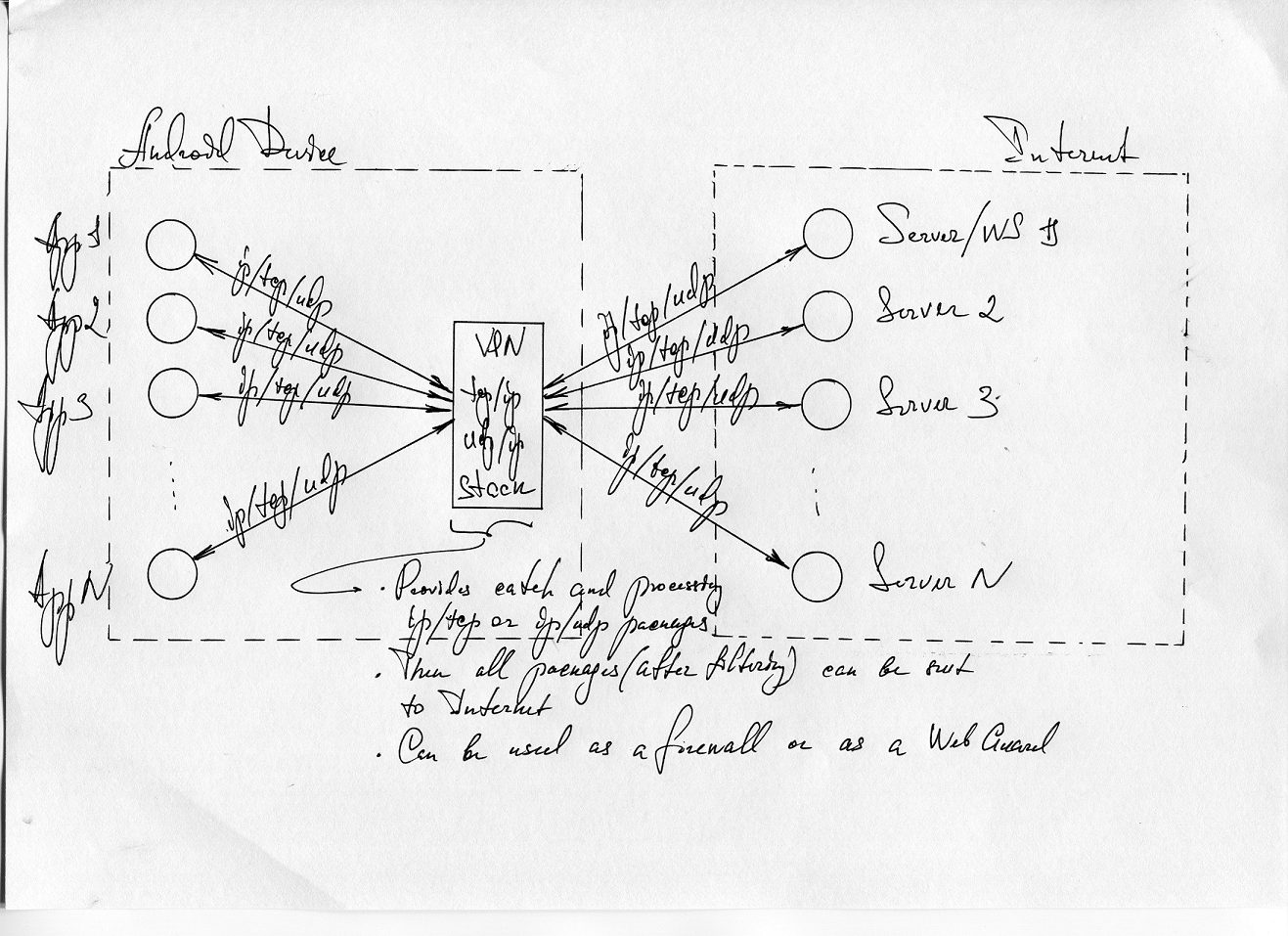Demo of the sm_vpn_stack_suite (Open-source Android VPN Stack Suite):
—————-

Here are results of the execution (log files and screenshots) of the socket_client_vs on Windows 10 x64.

socket_client_vs (Windows 10 x64)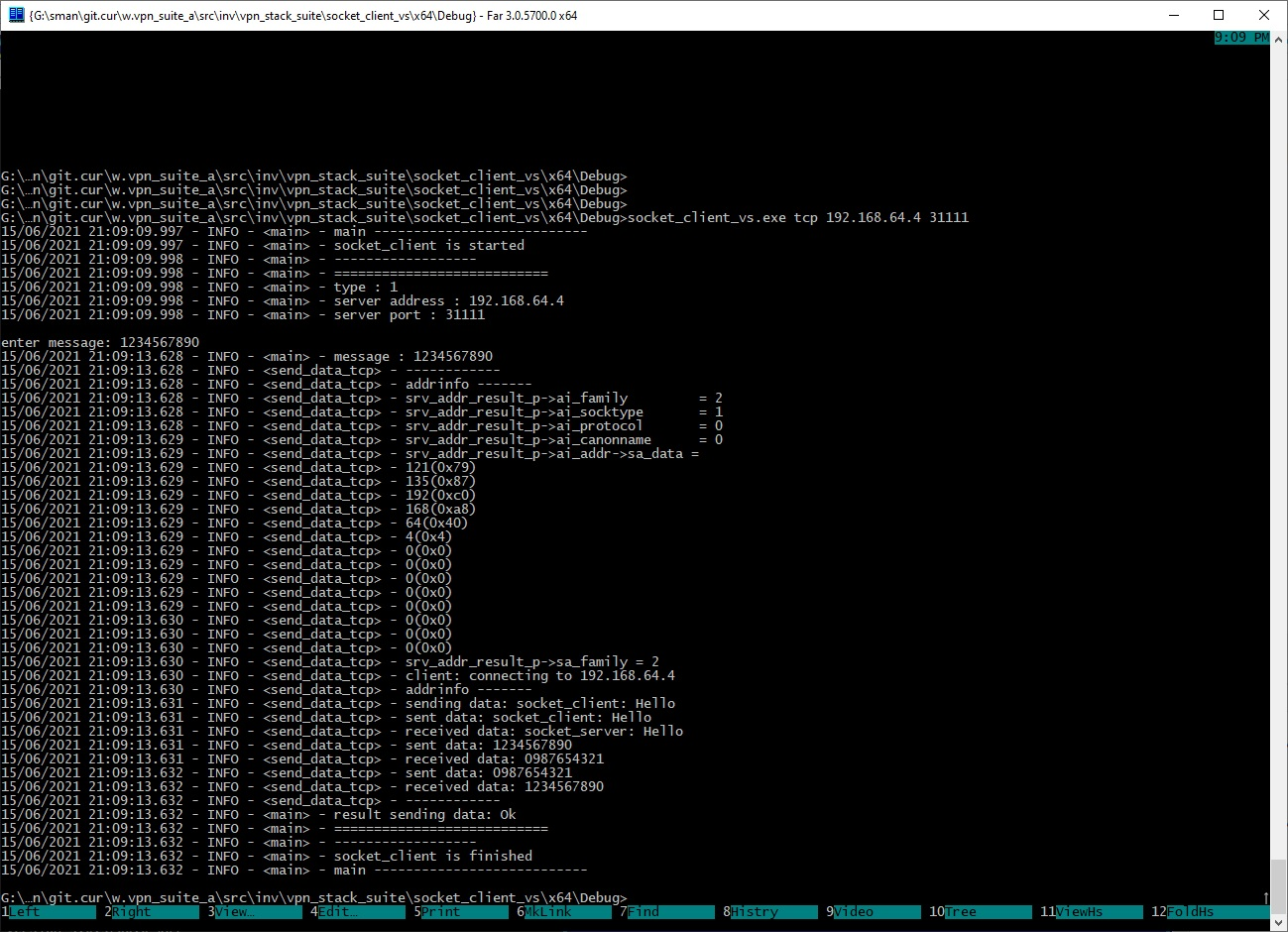Here are results of the execution  (log files and screenshots) of the socket_server on Linux Debian 10 x64.

Here are results of the execution (screenshot) of the socket_client on Android 8.0 (API 26) x64.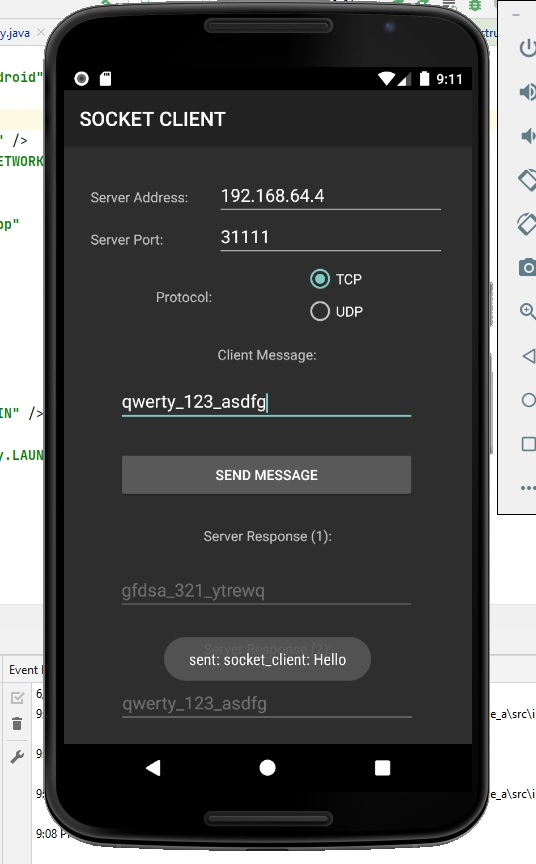Here are results of the execution  (log files and screenshots) of the vpn_stack on Android 8.0 (API 26) x64.

Here is list of network interfaces before launching the VPN Service.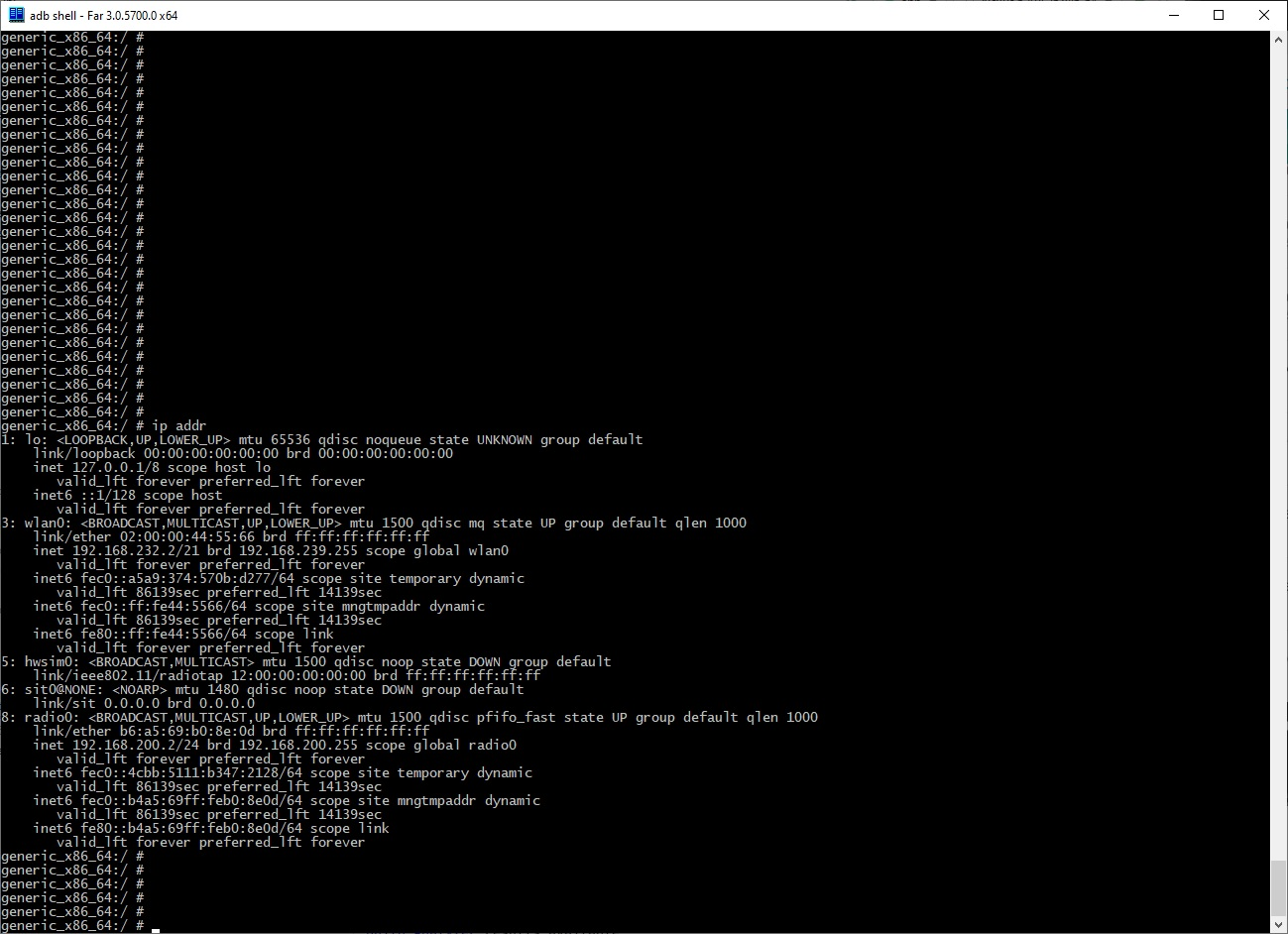Here is list of network interfaces after launching the VPN Service. Device tun0 (is used for catching the network traffic) has been added.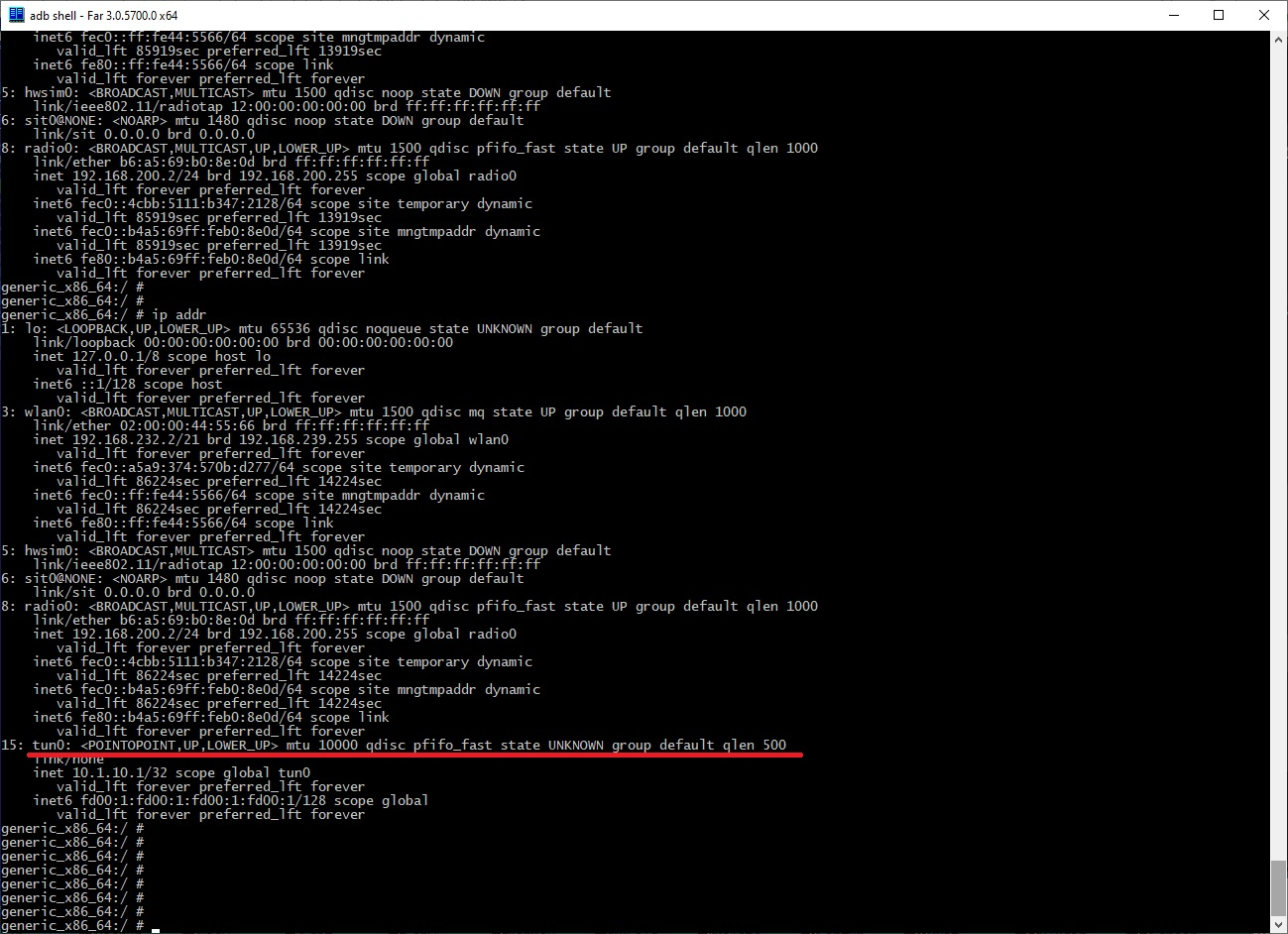Here are selected results (from vpn_stack.1.log and vpn_stack.2.log):

sm_vpn_stack_suite (Android VPN Stack Suite) 0.0.1.1 ® Copyright © 2021 by SManSoft.

Open-source multi-thread demo/template resolving the producer–consumer (bounded-buffer) problem (SM Producer Consumer) 0.0.1.1

Open-source multi-thread demo/template resolving the producer–consumer (bounded-buffer) problem (SM Producer Consumer) 0.0.1.1 has been released.

Supported platforms:

• Linux (x86_64)
• Examples (result file, log file), screenshots:
Examples

—————-

GPG Public Key (SManSoft ECDSA Key) <info@smansoft.com> has been published here: http://smansoft.com/gpg/smansoft.pub.asc.
SM Producer Consumer – 0.0.1.1 contains suite of [some_install_file].asc files.
gpg --import ./smansoft.pub.asc
for importing of key and
gpg --verify ./[some_install_file].asc ./[some_install_file]
or
gpg --verify ./[some_install_file].asc
for verification of files, published on this site.
Public GPG ECDSA Key can be imported, using
gpg --keyserver hkp://pgp.mit.edu --recv-keys A408B0FCFD774649
too.

Features of the SM Producer Consumer:
—————-

• Multi-thread demo/template, that resolves producer-consumer problem (bounded-buffer problem);
• Using thread support primitives (classes, api), which are parts of the C++ (C++11) language, defined by the standard ISO/IEC 14882:2011;
• Using several producer-consumer objects, which use suite of limited-size queues shared by various number of threads with various productivity/periodicity/frequency;
• Writing processed results into the text file (in the special directory);
• Logging results and applications workflow;
• Multi-thread (thread-safe) log library, that supports output to the log file and/or console;
• Multi-thread (thread-safe) log library, supports log level output (TRACE, DEBUG, INFO, WARN, ERROR, FATAL);
• Usage string secure library in some C-language functions;
• Self-stopping support, when file ‘.stop‘ is placed in the special directory;

Supported platforms:
—————-

Linux (x64 only):
• Debian 10;
• Debian 9;
• openSUSE 15.1;
• openSUSE 15.2;
• Ubuntu 20.04 LTS;
• Mint 19.3;
• Mint 20.1;

Demo of the sm_producer_consumer (SM Producer Consumer) tool (Linux):
—————-

Here are the results of the execution of the sm_producer_consumer (SM Producer Consumer) tool on Linux Debian 10 (Buster) x64.

Results of execution (result file, log file and screenshots) below:
sm_producer_consumer.log
sm_producer_consumer.out.txt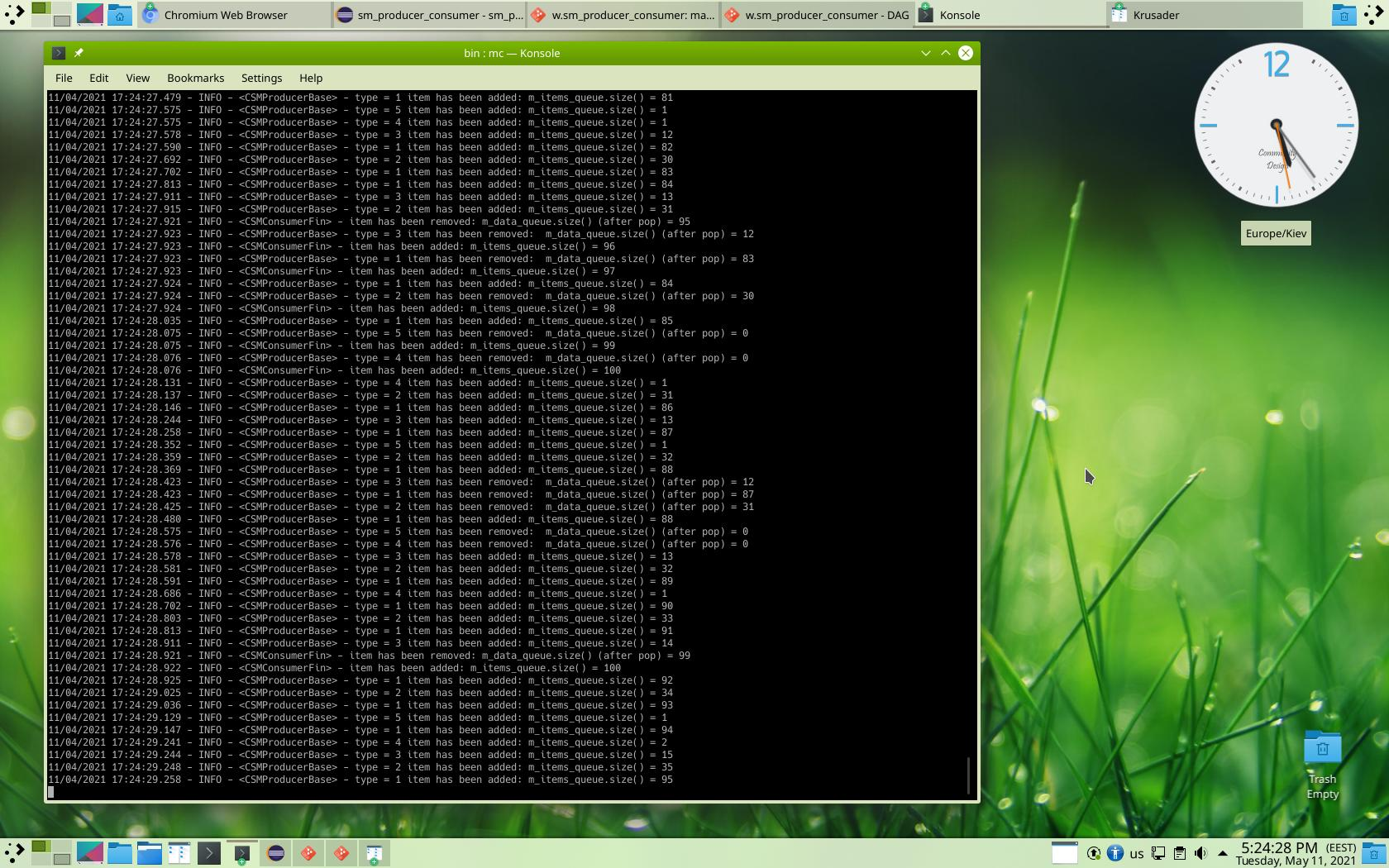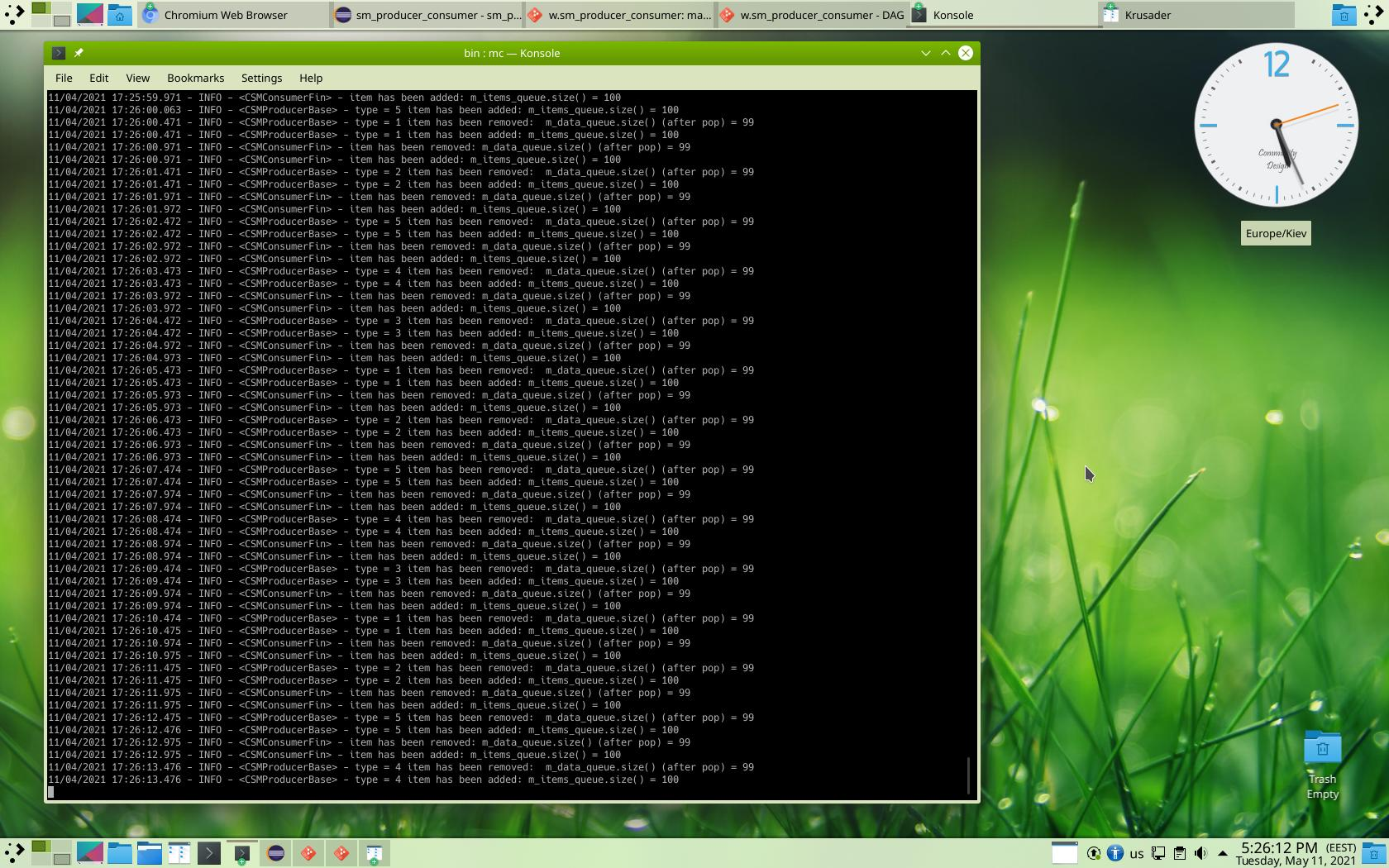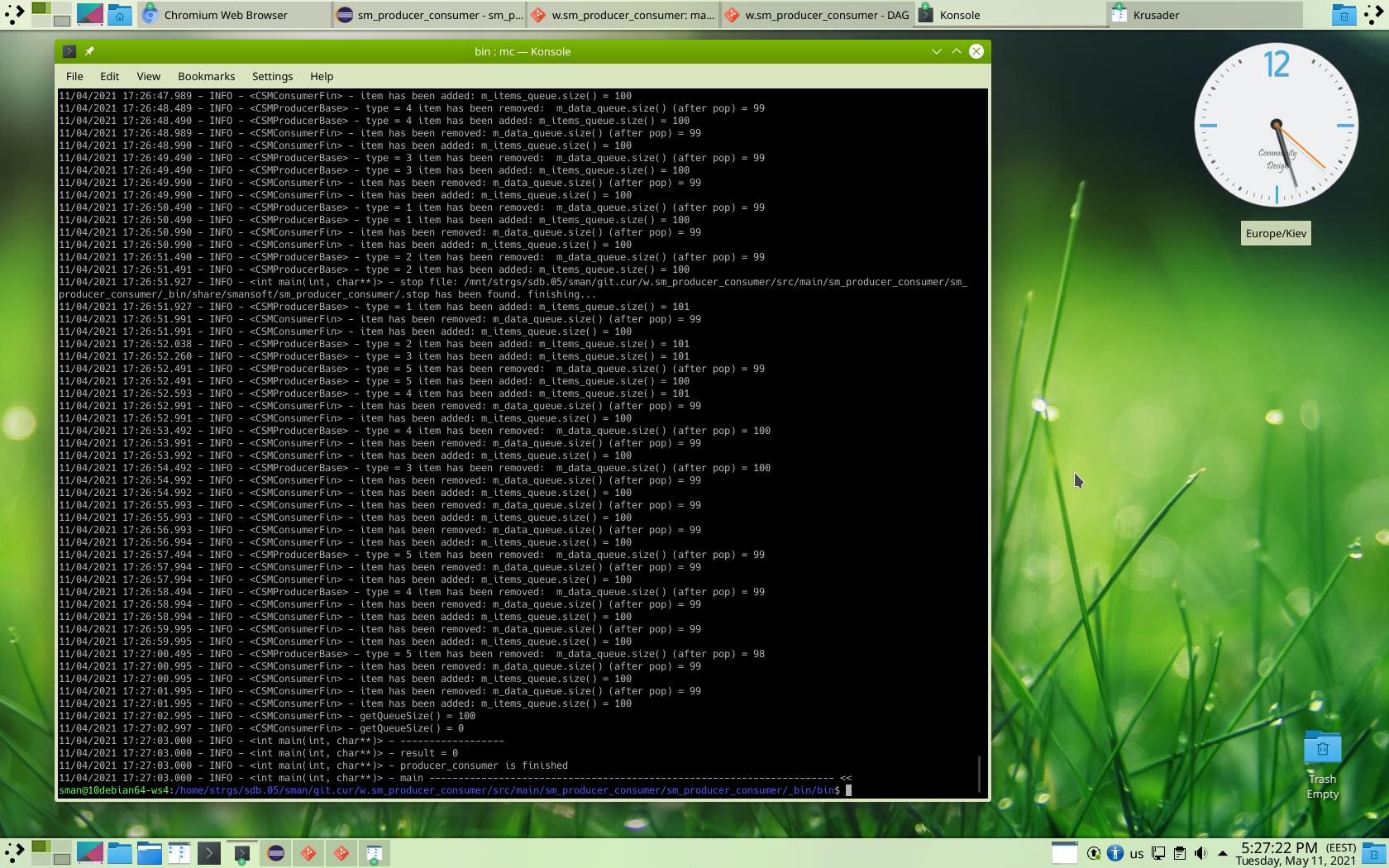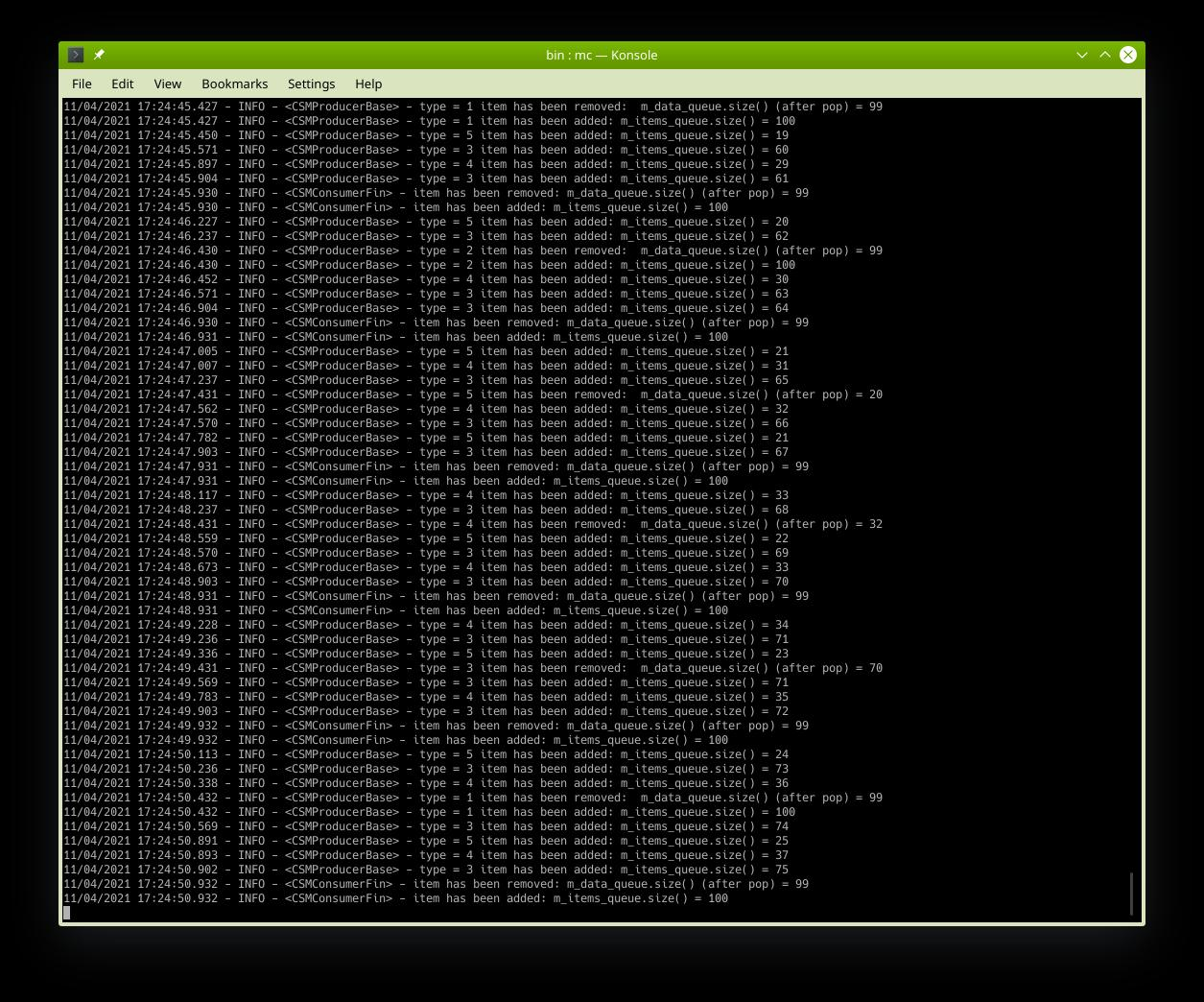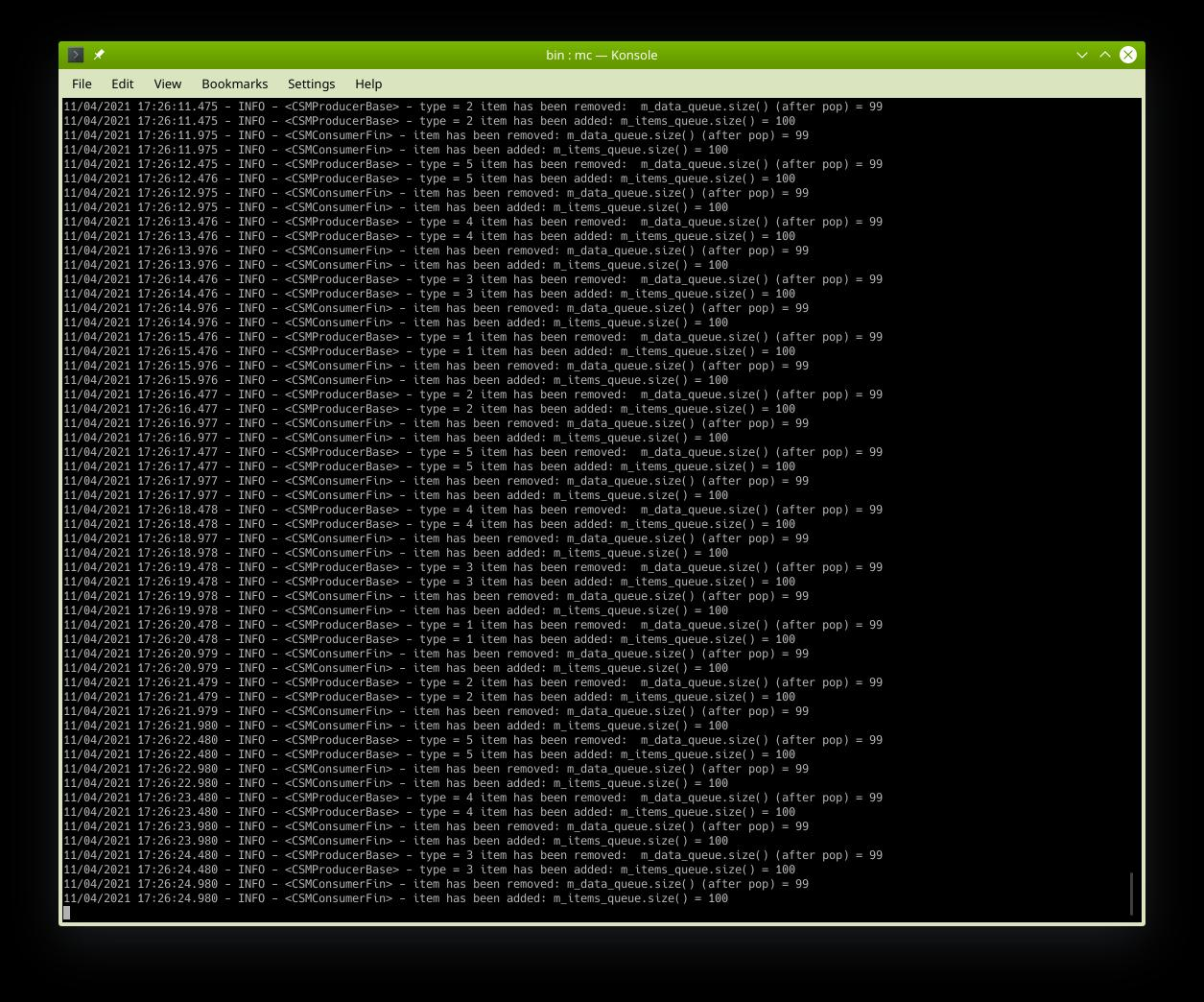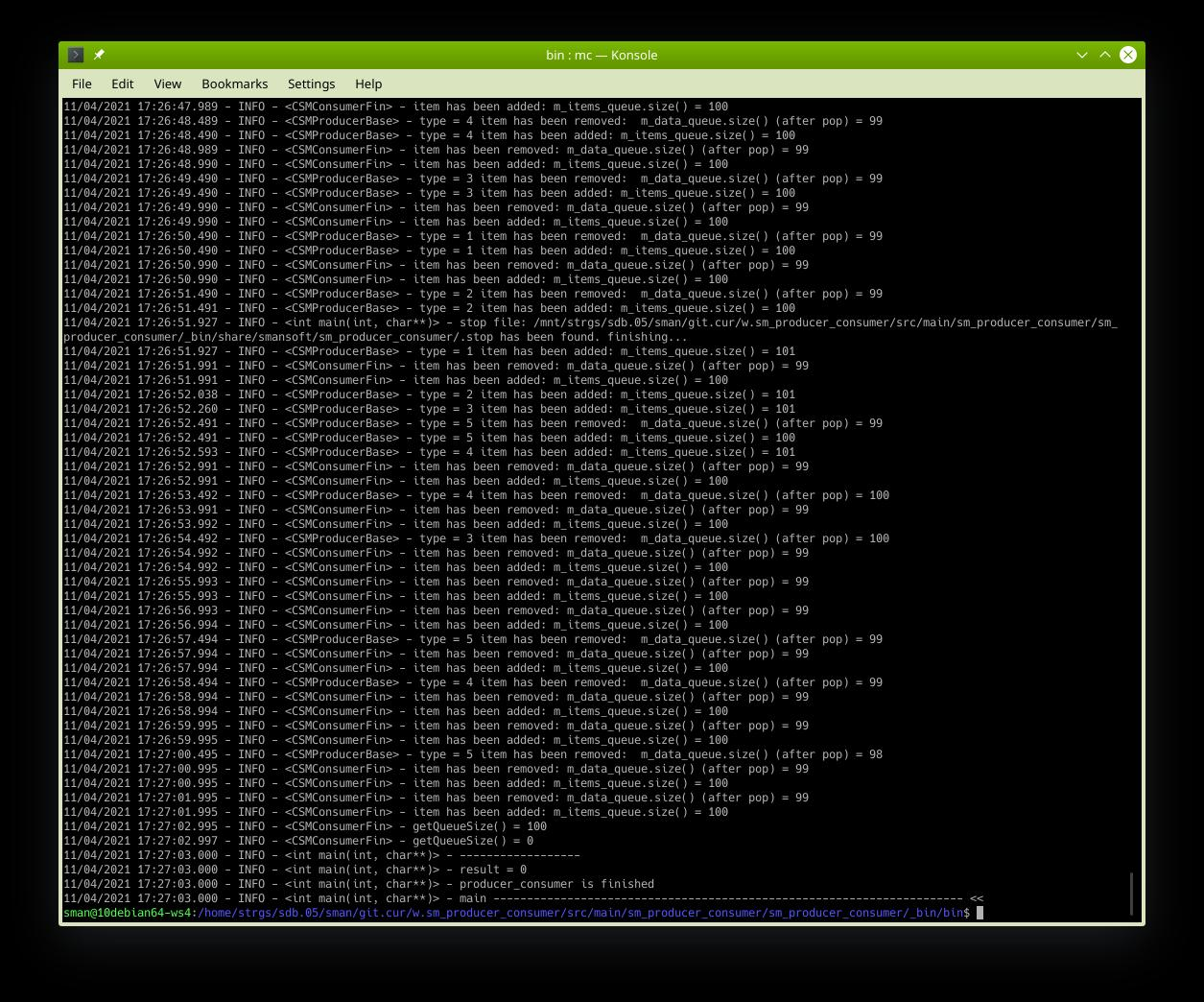Multi-Platform sm_regex (SM Regular Expressions Calculator) tool 0.0.1.1

Multi-Platform sm_regex (SM Regular Expressions Calculator) tool 0.0.1.1 has been released.

Supported platforms:

• Windows (x86, x86_64)
• Linux (x86_64)

—————-

GPG Public Key (SManSoft ECDSA Key) <info@smansoft.com> has been published here: http://smansoft.com/gpg/smansoft.pub.asc.
SM Regular Expressions Calculator – 0.0.1.1 contains suite of [some_install_file].asc files.
gpg --import ./smansoft.pub.asc
for importing of key and
gpg --verify ./[some_install_file].asc ./[some_install_file]
or
gpg --verify ./[some_install_file].asc
for verification of files, published on this site.
Public GPG ECDSA Key can be imported, using
gpg --keyserver hkp://pgp.mit.edu --recv-keys A408B0FCFD774649
too.

Features of the SM Regular Expressions Calculator:
—————-

• Multi-platform application: there are builds for Windows x64/x32 (.zip) and Linux x64 (.tar.gz);
• Calculation a Match (finds all substrings in the input string which match a regular expression pattern
and returns all occurrences) using regular expressions;
• Calculation a Replace (finds all substrings in the input string which match a regular expression pattern
and replaces all occurrences, by entered replacement text), using regular expressions;
• Calculation a SubExpressions (finds all substrings in the input string which match a regular expression pattern
and separates text, by found substrings), using regular expressions;
• Usage follow Regular Expressions engines: Qt RegEx, STL C++ RegEx (C++ 11);
• Usage ECMAScript standard (by both engines as Qt RegEx as STL C++ RegEx (C++ 11));
• One-line and multi-line modes;
• Case-insensitive and case-sensitive modes;
• Saving the result in the Clipboard;
• Saving the result in a Text File;
• Tuning interface, usage Light and Dark themes;
• Usage database, where Regular Expressions and options (one-line/multi-line and case-insensitive/case-sensitive), using during calculations can be saved;
• Usage the System Tray on the Windows and Linux platforms during work of the SM Regular Expressions Calculator tool;
• Log of results and applications workflow;
• Portable build/version (archive(s) which can be just extracted);

Supported platforms:
—————-

• Windows 7;
• Windows 8;
• Windows 8.1;
• Windows 10;
Linux (x64 only):
• Debian 10;
• Debian 9;
• openSUSE 15.1;
• openSUSE 15.2;
• Ubuntu 20.04 LTS;
• Mint 19.3;
• Mint 20.1;
• any x64 Linux distro, that uses version of glibc >= 2.24;

sm_regex (SM Regular Expressions Calculator) tool 0.0.1.1 ® Copyright © 2021 by SManSoft.

Multi-Platform Open-Source sm_calculator (SM Calculator) tool 0.2.0.4

Multi-Platform Open-Source sm_calculator (SM Calculator) tool 0.2.0.4 has been released.

New features of the SM Calculator (0.2.0.4):
—————-

• Multi-thread (reentrant) version of the parser;
• Unit tests for testing the multi-thread (reentrant) parser;
• Multi-thread synchronized mode is used in log_lib;
• Mutexes api from threads.h (C11 ISO/IEC 9899:2011) has been updated to mutexes api from pthread.h (POSIX);
• Some bugs have been fixed;

Supported platforms:

• Windows (x86, x86_64)
• Linux (x86, x86_64)
• Examples, the suite of sm_calc_test.in.XX.txt and sm_calc_test.out.XX.txt (also you can find the suite of sm_calc_test.in.XX.txt and sm_calc_test.out.XX.txt in directory /tests in sources):
Examples

—————-

GPG Public Key (SManSoft ECDSA Key) <info@smansoft.com> has been published here: http://smansoft.com/gpg/smansoft.pub.asc.
SM Calculator – 0.2.0.4 contains suite of [some_install_file].asc files.
gpg --import ./smansoft.pub.asc
for importing of key and
gpg --verify ./[some_install_file].asc ./[some_install_file]
or
gpg --verify ./[some_install_file].asc
for verification of files, published on this site.
Public GPG ECDSA Key can be imported, using
gpg --keyserver hkp://pgp.mit.edu --recv-keys A408B0FCFD774649
too.

Multi-Platform Open-Source sm_calculator (SM Calculator) tool 0.1.1.3

Multi-Platform Open-Source sm_calculator (SM Calculator) tool 0.1.1.3 has been released.

New features of the SM Calculator (0.1.1.3):
—————-

• Detailed processing of syntax error(s);
• Restoring of syntax parsing after error(s);

Supported platforms:

• Windows (x86, x86_64)
• Linux (x86, x86_64)
• Examples, the suite of sm_calc_test.in.XX.txt and sm_calc_test.out.XX.txt (also you can find the suite of sm_calc_test.in.XX.txt and sm_calc_test.out.XX.txt in directory /tests in sources):
Examples

—————-

GPG Public Key (SManSoft ECDSA Key) <info@smansoft.com> has been published here: http://smansoft.com/gpg/smansoft.pub.asc.
SM Calculator – 0.1.1.3 contains suite of [some_install_file].asc files.
gpg --import ./smansoft.pub.asc
for importing of key and
gpg --verify ./[some_install_file].asc ./[some_install_file]
or
gpg --verify ./[some_install_file].asc
for verification of files, published on this site.
Public GPG ECDSA Key can be imported, using
gpg --keyserver hkp://pgp.mit.edu --recv-keys A408B0FCFD774649
too.

Features of the sm_calculator (SM Calculator) tool 0.1.1.3:
—————-

• Multi-platform application: there are builds for Windows 64/32 (.zip) and Linux 64/32 (.tar.gz);
• Various types of values (64 bits integers, 64 bits floats, strings);
• Various float constants (pi, exp,…);
• Solving of mathematical expressions, using basic arithmetic operators;
• Solving of mathematical expressions, using bracket delimiters ‘(‘ and ‘)’ (in one expression) and delimiters ‘;’, ‘\n’;
• Solving using suite of mathematical (including trigonometric, logarithm, exponentiation) functions;
• Solving using bitwise functions and operators;
• Various types of entering and output formats of integers (decimal, octal, hexadecimal, binary formats);
• Various values of the unit for measuring of angles (radians, degrees, gradians), which are used by trigonometric functions;
• Support of output properties (precision) of float values;
• The suite of converting functions (various values of the unit for measuring of angles, various types of entering and output formats of integers);
• the suite of commands, which provide setup of the configuration of SM Calculator;
• Several modes of execution (processing of files, processing of expressions, obtained via command line, processing of expressions in interactive mode, using standard input/output devices);
• Control/administration the creation/start of the log of the sm_calculator (SM Calculator) tool:
command-line option: -q, --no_log
– changing the path of the created/opened log file:
command-line option: -l, --log_file;
• Access to the sm_calculator (SM Calculator) tool can be included into \$PATH (Windows/Linux) and the sm_calculator (SM Calculator) tool can be launched from any current directory;
• Detailed processing of syntax error(s);
• Restoring of syntax parsing after error(s);
• Help:
– command: help[;]
– command-line option: -h, --help;

SM Calculator tool supports the following modes of execution:
—————-

• processing of the file, that contains expression(s);
• processing of command-line expression(s);
• interactive mode (user enters expression(s), using standard input device/console).

A user can use the same expressions in all these modes (processing of the file,
processing of command-line expression(s), interactive mode). I.e. SM Calculator tool supports the same lexemes and grammar rules in all modes.

Limitations/disadvantages of the sm_calculator (SM Calculator) tool 0.1.1.3:
—————-

• sm_calculator (SM Calculator) tool 0.1.1.3  writes full log with detailed info without filtering of log messages by log level and without rolling of log files; So, a user should control the length of the log file by himself;
• sm_calculator (SM Calculator) tool 0.1.1.3 doesn’t guarantee correct execution when a user uses Unicode (UTF-16, partially UTF-8) encoding; sm_calculator (SM Calculator) tool 0.1.1.3 has been tested, for the usage of US-ASCII encoding symbols; Possible (according to cursory testing) user can use UTF-8 encoding (including national symbols) on Linux platform;

Structure of binary archive (.zip or .tar.gz):
—————-

• .zip (Windows):
bin\
sm_calc.exe
share\smansoft\doc\
HELP
CHANGELOG
• .tar.gz (Linux):
bin/
sm_calc
lib/
libgetopt_lib.so
share/smansoft/doc/
HELP
CHANGELOG

Execution:
—————-
sm_calc[.exe]
without parameters launches SM Calculator in interactive mode (default mode):

sm_calc 0.1.1.3 Copyright (c) 2020 SManSoft
Log has been started in the file: <path-to-log-dir>/sm_calc.log
SM Calculator
Interactive processing:
In >>

User can run:
sm_calc[.exe] -h
or
sm_calc[.exe] --help
to get help output – description of command-line parameters.

Using these options a user can launch SM Calculator in various modes of execution and tune start values of the current configuration of SM Calculator (command: config[;]):

-h, --help
-i, --interactive
-l<lfile>, --log_file=<lfile>
-q, --no_log
-f<infile>, --in_file=<infile>
-o<ofile>, --out_file=<ofile>
-x<expression_value>, --expression=<expression_value>
-p<precision_value>, --f_precision=<precision_value>
-n<i_format_value>, --i_format=<i_format_value>
-a<unit_value>, --a_unit=<unit_value>

Lexemes of SM Calculator expressions:
—————-

Available keywords:

Available operators:
+ - * / = ! <a< >a> <l< >l> <c< >c>

Available delimiters:
, ( ) ; \n (new line)

Commands (from available keywords):
f_precision i_format a_unit dec oct hex bin rad deg grad rand config help quit exit

Constants (from available keywords):
pi exp gamma phi

Output functions (from available keywords):
echo

Converting functions (from available keywords):

Trigonometric functions (from available keywords):
sin cos tan asin acos atan

Logarithm functions (from available keywords):
ln lg log

Exponentiation functions (from available keywords):
pow pow_exp^ pow_10^ pow_2^ pow_^2

Bit functions (from available keywords):
and or not nand nor xor

Bit operators (from available operators):
<a< >a> <l< >l> <c< >c>

Base syntax of commands:
—————-
command[;]
command=arg[;]

Base syntax of functions:
—————-
function (arg1[,arg2])[;]

Base syntax of operators:
—————-
arg1 operator arg1[;]
operator arg[;]

Operator assign (‘=’) can be used only in commands (f_precision, i_format, a_unit).

Types of values:
—————-

• Integer data (long long type – 64 bits);
• Float data (long double type – 64 bits);
• Strings (max length of string 1021 ASCII symbols);

Integer data:
—————-
Length of Integer data – 64 bits (including sign), i.e.
max integer value == 2^63-1;
min integer value == -(2^63-1);
Integer value can be entered and output in follow formats:

• decimal (‘dec‘);
• octal (‘oct‘);
• binary (‘bin‘);

The output format of integer value can be defined by command:
i_format = <dec|hex|oct|bin>[;]
A user can use shorter way for defining of the output format of an integer value, using commands:
dec[;]
hex[;]
oct[;]
bin[;]

The input format of an integer is defined by following rules:

• if a user enters integer value in octal format, he should use as least one lead ‘0’ symbol (for example: ‘0712377’, ‘-0712377’);
• if user enters integer value in hexadecimal format, he should use as lead ‘0x’ symbols (for example: ‘0x394ff’, ‘0xfffffffffffc6b01’);
• if user enters integer value in binary format, he should use as lead ‘b’ symbol (for example:  ‘b00111001010011111111’‘b1111111111111111111111111111111111111111111111000110101100000001’);
• if user enters integer value in decimal format, he shouldn’t use any additional symbols (for example: ‘129077’‘-129077’);

A user should take into account, that size of integer value is 64 bits (including sign), during entering value in various formats;

A user can enter negative values (in octal and hexadecimal) using as sign ‘-‘
as two’s complement format (see examples in the file ReadMe.txt):
-0712377 (oct) or 01777777777777777065401 (oct) == -234751 (dec)
-0x394ff (hex) or 0xfffffffffffc6b01 (hex) == -234751 (dec)

If user need to get current output format of integer values, he needs to use command:
i_format[;]

SM Calculator tool supports the suite of functions, which provide converting of various formats of integer values, whatever defined current output format of integer values:
dec2oct dec2hex dec2bin oct2dec hex2dec bin2dec.

Float data (long double – 64 bits):
—————-
The output format of float values always defined in decimal format. User can tune the precision of float value output, using the command:
f_precision = N[;]
, where N = [0,20]|exp, i.e. maximum precision of output of float value is ’20’.

Also, N can be defined as ‘exp’. In this case, the float value will be printed in an exponential format.

The input format of float values always defined in decimal format. A user can enter values as in floating form format as in exponential format.

If a user needs to get current output current precision of float, he needs to use the command:
f_precision[;].

Strings:
—————-
User should enter string value, using the following format:
"string_value" | 'string_value'.
Max length of the string – 1023 ASCII symbols with quotes(‘ or “)
or 1021 ASCII symbols without quotes(‘ or “).

String values aren’t used during calculations but could be used for the formatting of outputs in file processing, using the function
echo.

Default angle unit configuration:
—————-
SM calculator supports some suite of trigonometrical functions:
sin cos tan asin acos atan.
Also, SM calculator supports follow units for measuring of angles:

• degrees(‘deg‘)

SM Calculator tool contains the current configuration of the unit for measuring of angles. This parameter defines, which unit for measuring of angles will be used by trigonometric functions, i.e. if the current configuration of the unit for measuring of angles is radians, a user should use radians as parameters of trigonometric functions: sin(3.1415926536) or sin(pi), cos(1.5707963268) or cos(pi/2.0), if the current configuration of the unit for measuring of angles is degrees, a user should use degrees as parameters of trigonometric functions: sin(180.0), cos(90), same issue with the usage of gradians.
The current configuration of the unit for measuring of angles can be defined by the following command:

Also, user can setup current configuration of the unit for measuring of angles, just using following commands:
deg[;]

User can get current configuration of the unit for measuring of angles using the command:
a_unit[;]

SM Calculator tool supports the suite of functions, which provide converting of the various unit for measuring of angles, whatever defined current configuration of the unit for measuring:

Current configuration:
—————-
A user always can get current configuration, using the command:
config[;]
The result of the command config[;]:
Current Configuration :
---------------------------------------------
Current double precision : EXPONENTIAL;
Current output format of integers : dec;
Unit of measurement of an angle : rad;

Constants:
—————-
SM Calculator supports follow float (64 bits) constants:
pi
exp
gamma
phi

Constants return float values. They can be used in expressions as arguments of functions and operators.

Examples:
—————-
User can find here suite of files which contain suite examples of usage of operators, functions, commands,
constants, various types of data:

Examples

 Examples of expressions: Results of execution of expressions: sm_calc_test.in.01.txt sm_calc_test.out.01.txt sm_calc_test.in.02.txt sm_calc_test.out.02.txt sm_calc_test.in.03.txt sm_calc_test.out.03.txt sm_calc_test.in.04.txt sm_calc_test.out.04.txt sm_calc_test.in.05.txt sm_calc_test.out.05.txt sm_calc_test.in.06.txt sm_calc_test.out.06.txt sm_calc_test.in.07.txt sm_calc_test.out.07.txt sm_calc_test.in.08.txt sm_calc_test.out.08.txt

Also, Examples contains:

• screenshots (.jpeg);
• video demo (.mp4);
• demo log files (.log);

—————-

Help.txt:
—————-
The short reference of commands, constants, functions, operators of the sm_calculator (SM Calculator) tool 0.1.1.3 is published here:
Help.txt

Demo of the sm_calculator (SM Calculator) tool 0.1.1.3 (Windows):
—————-

Here are the results of the execution of the sm_calculator (SM Calculator) tool 0.1.1.3 on Microsoft Windows 10 x64.

The sm_calculator (SM Calculator) tool 0.1.1.3 has been launched 4 times:

1. sm_calc --help
2. sm_calc --expression="pow_^2(sin(pi/2))+pow_^2(cos(pi/2));"
3. sm_calc --in_file="./sm_calc_test.in.01.txt" --out_file="./sm_calc_test.out.01.txt"
4. sm_calc

Results of execution (log file and screenshots) below:
sm_calc.log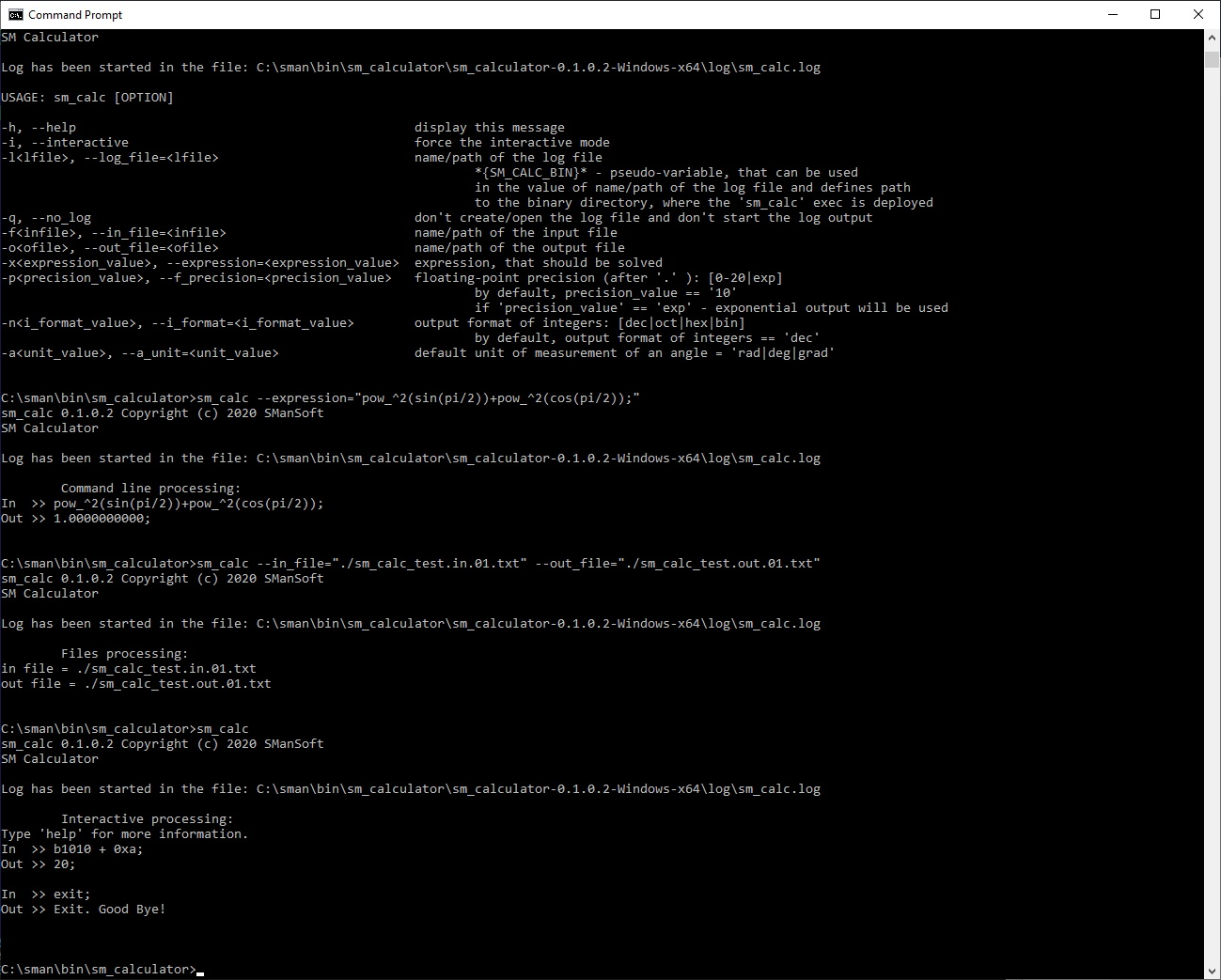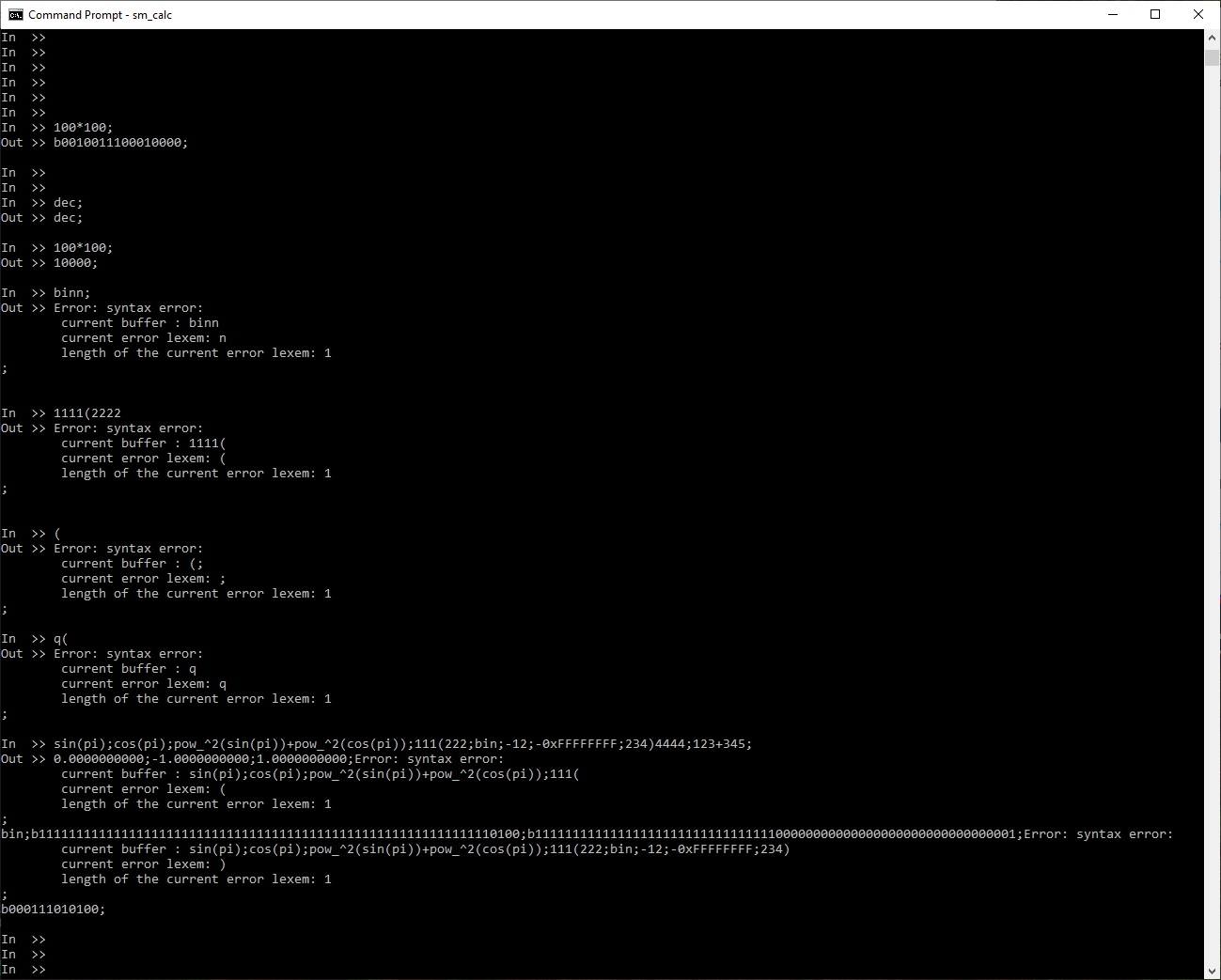Demo of the sm_calculator (SM Calculator) tool 0.1.1.3 (Linux):
—————-

Here are the results of the execution of the sm_calculator (SM Calculator) tool 0.1.1.3 on Linux Debian 10 (Buster) x64.

The sm_calculator (SM Calculator) tool 0.1.1.3 has been launched 4 times:

1. sm_calc --help
2. sm_calc --expression="pow_^2(sin(pi/2))+pow_^2(cos(pi/2));"
3. sm_calc --in_file="./sm_calc_test.in.02.txt" --out_file="./sm_calc_test.out.02.txt"
4. sm_calc

Results of execution (log file and screenshots) below:
sm_calc.log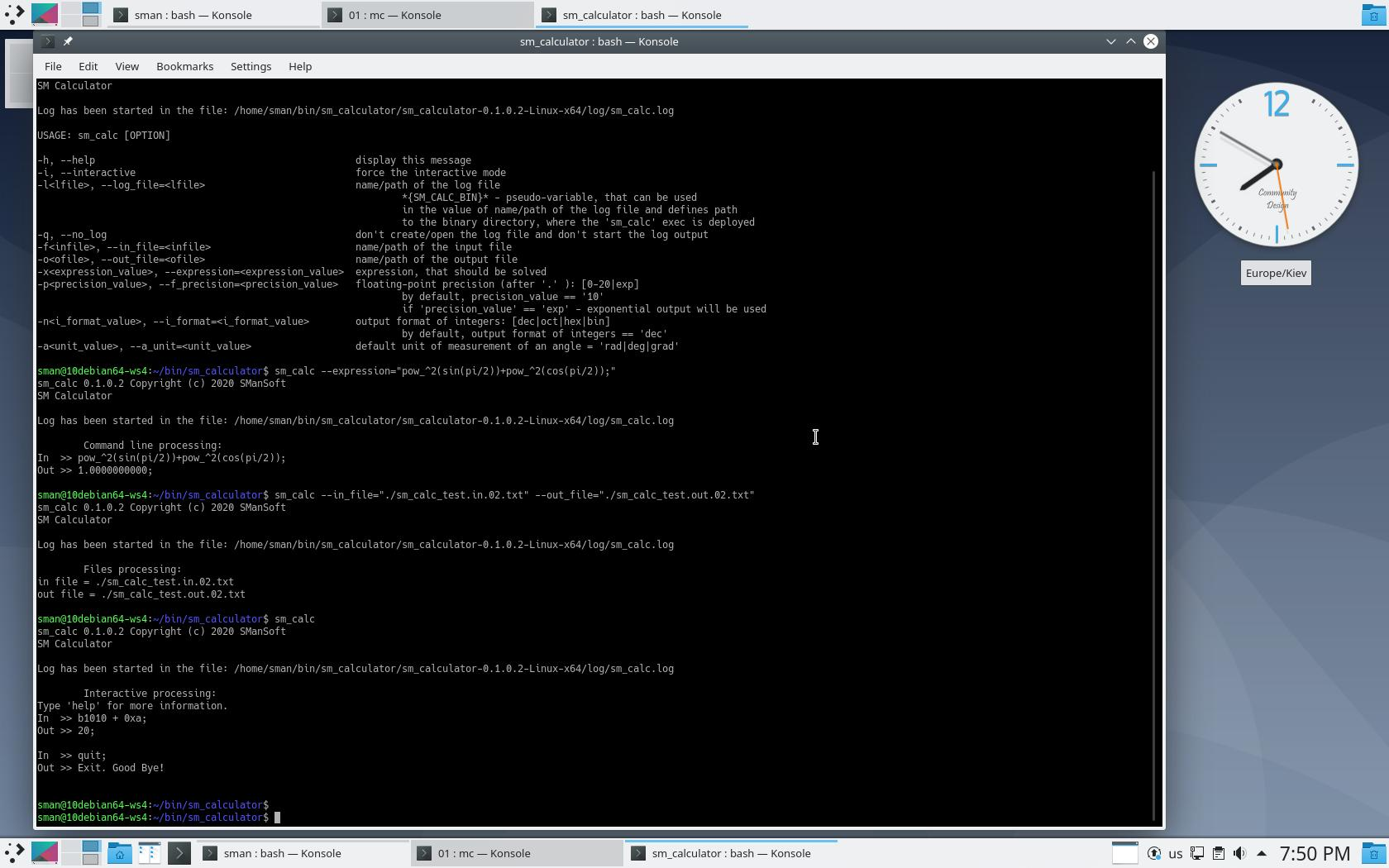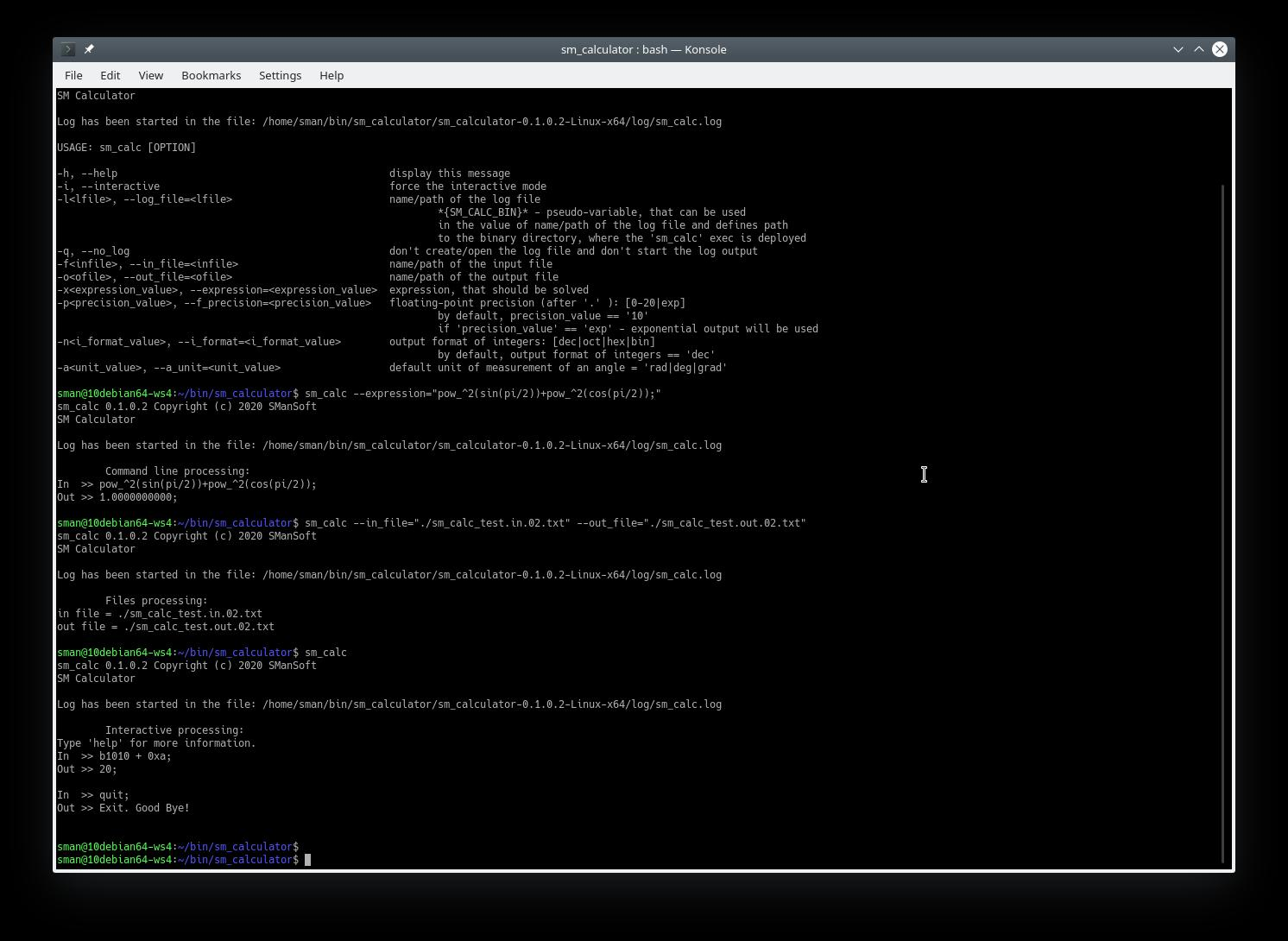—————-

Building the sm_calculator (SM Calculator) solution 0.1.0.2

Description of sources, structure, third-party tools, and assembling the Multi-Platform Open-Source sm_calculator (SM Calculator) solution 0.1.0.2.

—————-

Source files of the sm_calculator (SM Calculator)
can be found here:
SM Calculator – 0.1.0.2
sm_calculator-0.1.0.2-Source.zip
sm_calculator-0.1.0.2-Source.tar.gz

Also, you can find source files here:
sm_calculator on GitHub

You can use the command:
git clone https://github.com/smansoft/sm_calculator.git
to get sources from the GitHub repository.

Install.txt
—————-
Here is a detailed description of the solution, sources, necessary tools, and ways, how the sm_calculator (SM Calculator) solution 0.1.0.2 can be built and tested:
Install.txt.

Third-party libraries and tools
—————-
You can find a suite of third-party libraries and tools here (SM Calculator – 0.1.0.2): cmocka, getopt, safestringlib, win_flex_bison (Install.txt). Here are:

• win_flex_bison – windows port of Flex and Bison tools
• getopt – library (adopted for Visual Studio) for parsing of command-line aruments
• safestringlib – a multi-platform library, that contains API for safe control of memory and strings
• cmocka – unit test library

You can download win_flex_bison for assembling the sm_calculator (SM Calculator) solution 0.1.0.2 on Windows and you don’t need other third-party libraries for assembling the sm_calculator (SM Calculator) solution 0.1.0.2 as they are already included in sources (/deps).

Tools necessary for assembling of the project:
—————-

Windows platform:

• Visual Studio 2019 (including NMake tool);
• CMake;
• flex;
• bison;
• diff (optionally);
• Python 3 (optionally);

Linux platform:

• GCC;
• Make tool;
• CMake;
• flex;
• bison;
• bash;
• diff (optionally);
• Python 3 (optionally);

Some issue of assembling on the Linux platform, using synchronized log (definition: SM_SYNC_LOG):
—————-

The sm_calculator (SM Calculator) solution 0.1.0.2 contains project log_lib, which contains the support of synchronized print (definition: SM_SYNC_LOG). As a rule, there is no necessity of usage of synchronizing print, as every log print is executed by one call of runtime the function fprintf  of synchronized C-runtime API. But there are some cases when this synchronizing can de used (Install.txt). Windows tree of sources uses standard critical section objects in synchronizing API. Linux tree of sources uses thread API, defined by C11 (ISO/IEC 9899:2011) standard, using #include <threads.h>. But not all packages of GCC contain the support of the thread API, defined by C11 (threads.h), though GCC supports the C11 standard. This problem can be resolved after updating of usage of the thread API, defined by C11 (#include <threads.h>) to the usage of the POSIX thread API (#include <pthread.h>).

Build of the sm_calculator (SM Calculator) solution 0.1.0.2 (Windows):
—————-

Here is a build screenshot, using
/_cmake/cmake_build.nmake.bat
See Install.txt for more details about the way of a build of the solution, using /_cmake/cmake_build.nmake.bat. /_cmake – means subdirectory _cmake of the root of sources of the sm_calculator (SM Calculator) solution 0.1.0.2.

Build of the sm_calculator (SM Calculator) solution 0.1.0.2 includes following steps:

• creating of the subdirectory /_cmake/_build
• cmake -G "NMake Makefiles" -DCMAKE_BUILD_TYPE="Release" "..\.." – generating of necessary project/build files, including Makefile for the NMake tool
• nmake clean – clean target in Makefile (generated by CMake)
• nmake – build of the solution
• nmake test – launching unit tests
• cpack -G "ZIP" – generating pack archive, that contains binaries (.zip)
• cpack -G "ZIP" --config CPackSourceConfig.cmake – generating pack archive, that contains sources (.zip)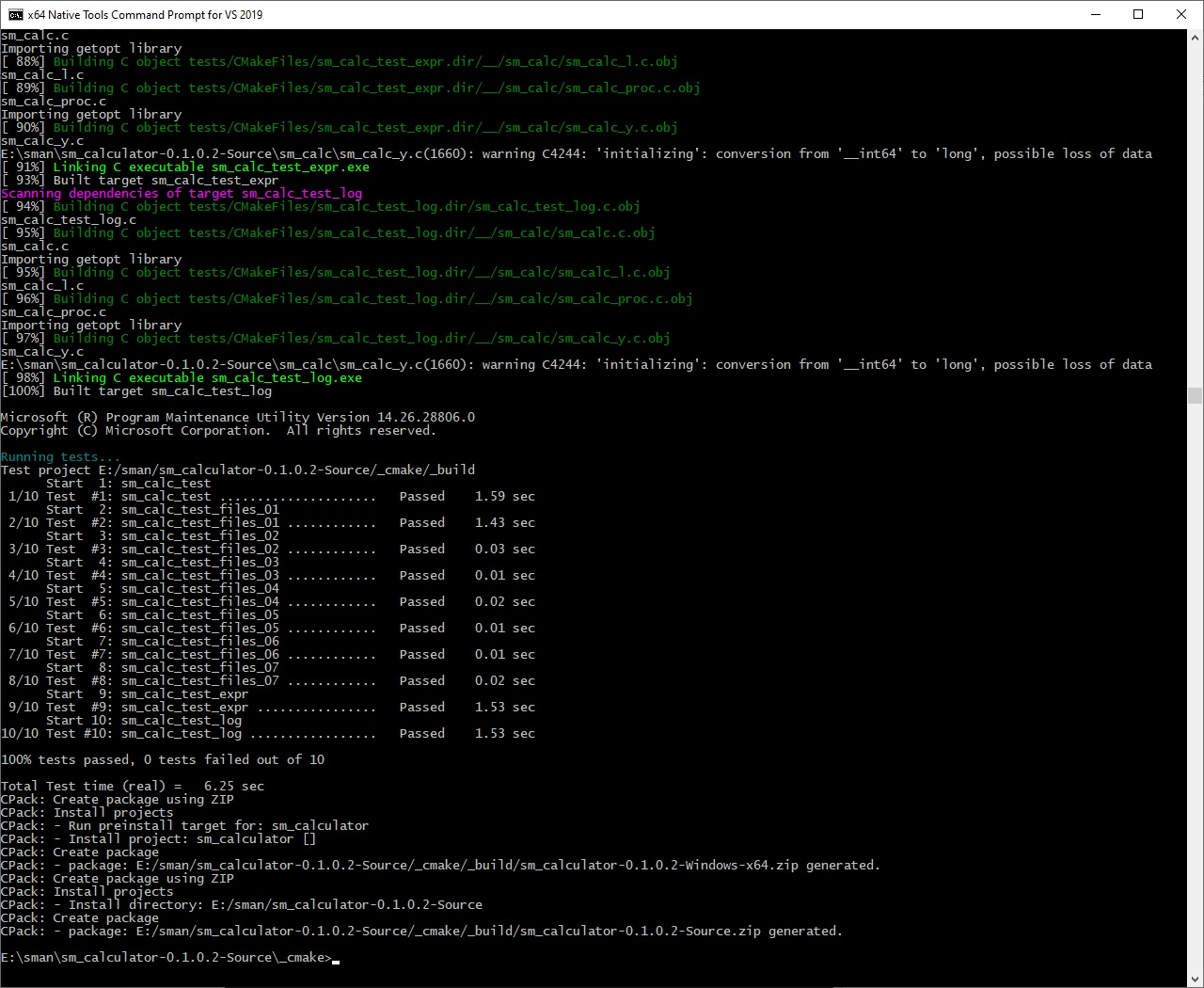Build of the sm_calculator (SM Calculator) solution 0.1.0.2 (Linux):
—————-

Here is a build screenshot, using
/_cmake/cmake_build.sh
See Install.txt for more details about the way of a build of the solution, using /_cmake/cmake_build.sh.

Build of the sm_calculator (SM Calculator) solution 0.1.0.2 includes following steps:

• creating of the subdirectory /_cmake/_build
• cmake -G "Unix Makefiles" -DCMAKE_BUILD_TYPE="Release" "../.."" – generating of necessary project/build files, including Makefile for the Make tool
• make clean – clean target in Makefile (generated by CMake)
• make all test – build of the solution and launching unit tests
• cpack -G "TGZ" – generating pack archive, that contains binaries (.tar.gz)
• cpack -G "TGZ" --config CPackSourceConfig.cmake – generating pack archive, that contains sources (.tar.gz)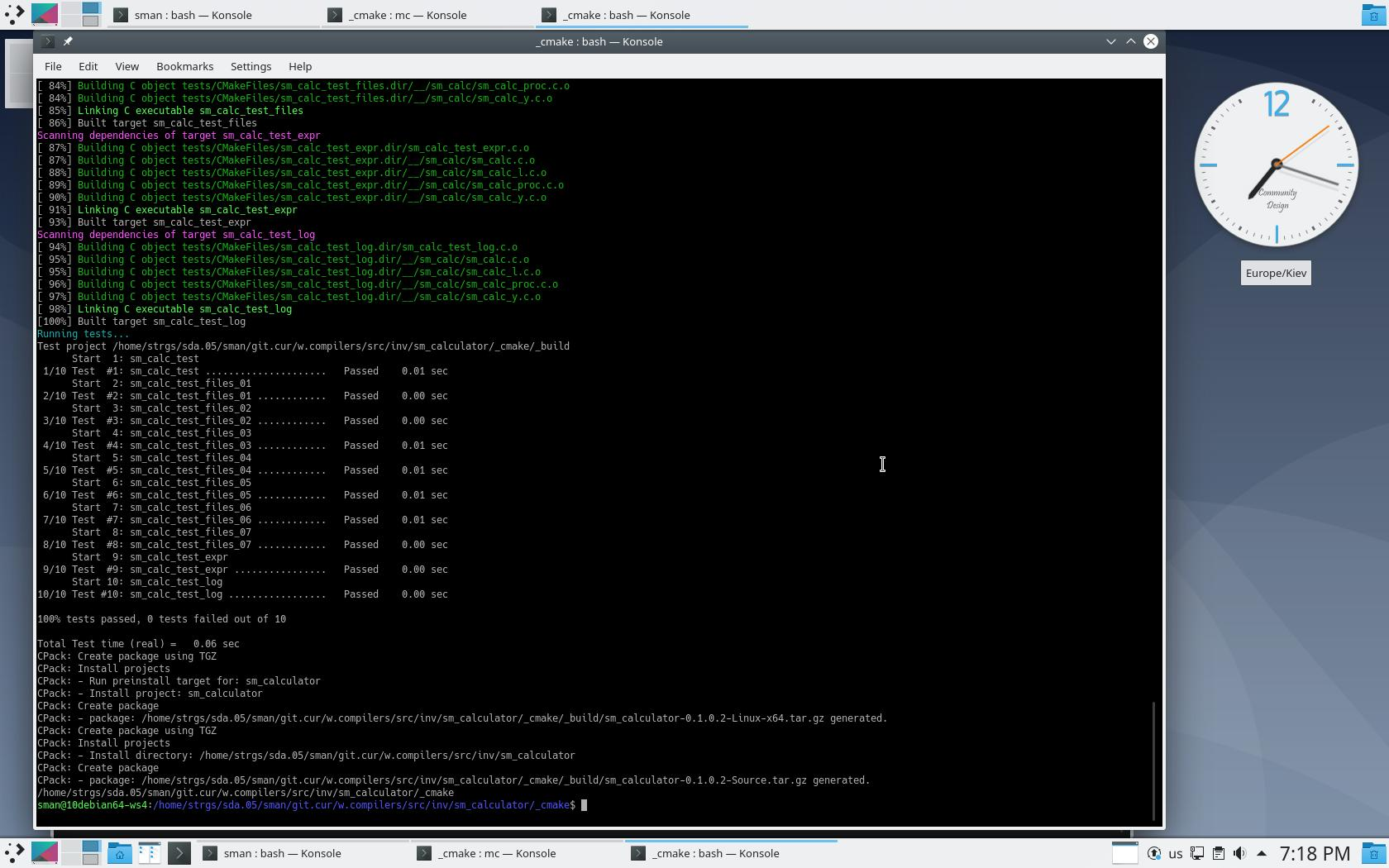—————-

Multi-Platform Open-Source sm_calculator (SM Calculator) tool 0.1.0.2

Multi-Platform Open-Source sm_calculator (SM Calculator) tool 0.1.0.2 has been released.

New features of the SM Calculator (0.1.0.2):
—————-

• Control/administration the creation/start of the log of the sm_calculator (SM Calculator) tool:
command-line option: -q, --no_log
– changing the path of the created/opened log file:
command-line option: -l, --log_file;
• Access to the sm_calculator (SM Calculator) tool can be included into \$PATH (Windows/Linux) and the sm_calculator (SM Calculator) tool can be launched from any current directory;

Supported platforms:

• Windows (x86, x86_64)
• Linux (x86, x86_64)
• Examples, the suite of sm_calc_test.in.XX.txt and sm_calc_test.out.XX.txt (also you can find the suite of sm_calc_test.in.XX.txt and sm_calc_test.out.XX.txt in directory /tests in sources):
Examples

—————-

GPG Public Key (SManSoft ECDSA Key) <info@smansoft.com> has been published here: http://smansoft.com/gpg/smansoft.pub.asc.
SM Calculator – 0.1.0.2 contains suite of [some_install_file].asc files.
gpg --import ./smansoft.pub.asc
for importing of key and
gpg --verify ./[some_install_file].asc ./[some_install_file]
or
gpg --verify ./[some_install_file].asc
for verification of files, published on this site.
Public GPG ECDSA Key can be imported, using
gpg --keyserver hkp://pgp.mit.edu --recv-keys A408B0FCFD774649
too.

Features of the sm_calculator (SM Calculator) tool 0.1.0.2:
—————-

• Multi-platform application: there are builds for Windows 64/32 (.zip) and Linux 64/32 (.tar.gz);
• Various types of values (64 bits integers, 64 bits floats, strings);
• Various float constants (pi, exp,…);
• Solving of mathematical expressions, using basic arithmetic operators;
• Solving of mathematical expressions, using bracket delimiters ‘(‘ and ‘)’ (in one expression) and delimiters ‘;’, ‘\n’;
• Solving using suite of mathematical (including trigonometric, logarithm, exponentiation) functions;
• Solving using bitwise functions and operators;
• Various types of entering and output formats of integers (decimal, octal, hexadecimal, binary formats);
• Various values of the unit for measuring of angles (radians, degrees, gradians), which are used by trigonometric functions;
• Support of output properties (precision) of float values;
• The suite of converting functions (various values of the unit for measuring of angles, various types of entering and output formats of integers);
• the suite of commands, which provide setup of the configuration of SM Calculator;
• Several modes of execution (processing of files, processing of expressions, obtained via command line, processing of expressions in interactive mode, using standard input/output devices);
• Control/administration the creation/start of the log of the sm_calculator (SM Calculator) tool:
command-line option: -q, --no_log
– changing the path of the created/opened log file:
command-line option: -l, --log_file;
• Access to the sm_calculator (SM Calculator) tool can be included into \$PATH (Windows/Linux) and the sm_calculator (SM Calculator) tool can be launched from any current directory;
• Help:
– command: help[;]
– command-line option: -h, --help;

SM Calculator tool supports the following modes of execution:
—————-

• processing of the file, that contains expression(s);
• processing of command-line expression(s);
• interactive mode (user enters expression(s), using standard input device/console).

A user can use the same expressions in all these modes (processing of the file,
processing of command-line expression(s), interactive mode). I.e. SM Calculator tool supports the same lexemes and grammar rules in all modes.

Limitations/disadvantages of the sm_calculator (SM Calculator) tool 0.1.0.2:
—————-

• sm_calculator (SM Calculator) tool 0.1.0.2  writes full log with detailed info without filtering of log messages by log level and without rolling of log files; So, a user should control the length of the log file by himself;
• sm_calculator (SM Calculator) tool 0.1.0.2 doesn’t guarantee correct execution when a user uses Unicode (UTF-16, partially UTF-8) encoding; sm_calculator (SM Calculator) tool 0.1.0.2 has been tested, for the usage of US-ASCII encoding symbols; Possible (according to cursory testing) user can use UTF-8 encoding (including national symbols) on Linux platform;

Structure of binary archive (.zip or .tar.gz):
—————-

• .zip (Windows):
bin\
sm_calc.exe
share\smansoft\doc\
HELP
CHANGELOG
• .tar.gz (Linux):
bin/
sm_calc
lib/
libgetopt_lib.so
share/smansoft/doc/
HELP
CHANGELOG

Execution:
—————-
sm_calc[.exe]
without parameters launches SM Calculator in interactive mode (default mode):

sm_calc 0.1.0.2 Copyright (c) 2020 SManSoft
Log has been started in the file: <path-to-log-dir>/sm_calc.log
SM Calculator
Interactive processing:
In >>

User can run:
sm_calc[.exe] -h
or
sm_calc[.exe] --help
to get help output – description of command-line parameters.

Using these options a user can launch SM Calculator in various modes of execution and tune start values of the current configuration of SM Calculator (command: config[;]):

-h, --help
-i, --interactive
-l<lfile>, --log_file=<lfile>
-q, --no_log
-f<infile>, --in_file=<infile>
-o<ofile>, --out_file=<ofile>
-x<expression_value>, --expression=<expression_value>
-p<precision_value>, --f_precision=<precision_value>
-n<i_format_value>, --i_format=<i_format_value>
-a<unit_value>, --a_unit=<unit_value>

Lexemes of SM Calculator expressions:
—————-

Available keywords:

Available operators:
+ - * / = ! <a< >a> <l< >l> <c< >c>

Available delimiters:
, ( ) ; \n (new line)

Commands (from available keywords):
f_precision i_format a_unit dec oct hex bin rad deg grad rand config help quit exit

Constants (from available keywords):
pi exp gamma phi

Output functions (from available keywords):
echo

Converting functions (from available keywords):

Trigonometric functions (from available keywords):
sin cos tan asin acos atan

Logarithm functions (from available keywords):
ln lg log

Exponentiation functions (from available keywords):
pow pow_exp^ pow_10^ pow_2^ pow_^2

Bit functions (from available keywords):
and or not nand nor xor

Bit operators (from available operators):
<a< >a> <l< >l> <c< >c>

Base syntax of commands:
—————-
command[;]
command=arg[;]

Base syntax of functions:
—————-
function (arg1[,arg2])[;]

Base syntax of operators:
—————-
arg1 operator arg1[;]
operator arg[;]

Operator assign (‘=’) can be used only in commands (f_precision, i_format, a_unit).

Types of values:
—————-

• Integer data (long long type – 64 bits);
• Float data (long double type – 64 bits);
• Strings (max length of string 1021 ASCII symbols);

Integer data:
—————-
Length of Integer data – 64 bits (including sign), i.e.
max integer value == 2^63-1;
min integer value == -(2^63-1);
Integer value can be entered and output in follow formats:

• decimal (‘dec‘);
• octal (‘oct‘);
• binary (‘bin‘);

The output format of integer value can be defined by command:
i_format = <dec|hex|oct|bin>[;]
A user can use shorter way for defining of the output format of an integer value, using commands:
dec[;]
hex[;]
oct[;]
bin[;]

The input format of an integer is defined by following rules:

• if a user enters integer value in octal format, he should use as least one lead ‘0’ symbol (for example: ‘0712377’, ‘-0712377’);
• if user enters integer value in hexadecimal format, he should use as lead ‘0x’ symbols (for example: ‘0x394ff’, ‘0xfffffffffffc6b01’);
• if user enters integer value in binary format, he should use as lead ‘b’ symbol (for example:  ‘b00111001010011111111’‘b1111111111111111111111111111111111111111111111000110101100000001’);
• if user enters integer value in decimal format, he shouldn’t use any additional symbols (for example: ‘129077’‘-129077’);

A user should take into account, that size of integer value is 64 bits (including sign), during entering value in various formats;

A user can enter negative values (in octal and hexadecimal) using as sign ‘-‘
as two’s complement format (see examples in the file ReadMe.txt):
-0712377 (oct) or 01777777777777777065401 (oct) == -234751 (dec)
-0x394ff (hex) or 0xfffffffffffc6b01 (hex) == -234751 (dec)

If user need to get current output format of integer values, he needs to use command:
i_format[;]

SM Calculator tool supports the suite of functions, which provide converting of various formats of integer values, whatever defined current output format of integer values:
dec2oct dec2hex dec2bin oct2dec hex2dec bin2dec.

Float data (long double – 64 bits):
—————-
The output format of float values always defined in decimal format. User can tune the precision of float value output, using the command:
f_precision = N[;]
, where N = [0,20]|exp, i.e. maximum precision of output of float value is ’20’.

Also, N can be defined as ‘exp’. In this case, the float value will be printed in an exponential format.

The input format of float values always defined in decimal format. A user can enter values as in floating form format as in exponential format.

If a user needs to get current output current precision of float, he needs to use the command:
f_precision[;].

Strings:
—————-
User should enter string value, using the following format:
"string_value" | 'string_value'.
Max length of the string – 1023 ASCII symbols with quotes(‘ or “)
or 1021 ASCII symbols without quotes(‘ or “).

String values aren’t used during calculations but could be used for the formatting of outputs in file processing, using the function
echo.

Default angle unit configuration:
—————-
SM calculator supports some suite of trigonometrical functions:
sin cos tan asin acos atan.
Also, SM calculator supports follow units for measuring of angles:

• degrees(‘deg‘)

SM Calculator tool contains the current configuration of the unit for measuring of angles. This parameter defines, which unit for measuring of angles will be used by trigonometric functions, i.e. if the current configuration of the unit for measuring of angles is radians, a user should use radians as parameters of trigonometric functions: sin(3.1415926536) or sin(pi), cos(1.5707963268) or cos(pi/2.0), if the current configuration of the unit for measuring of angles is degrees, a user should use degrees as parameters of trigonometric functions: sin(180.0), cos(90), same issue with the usage of gradians.
The current configuration of the unit for measuring of angles can be defined by the following command:

Also user can setup current configuration of the unit for measuring of angles, just using following commands:
deg[;]

User can get current configuration of the unit for measuring of angles using the command:
a_unit[;]

SM Calculator tool supports the suite of functions, which provide converting of the various unit for measuring of angles, whatever defined current configuration of the unit for measuring:

Current configuration:
—————-
A user always can get current configuration, using the command:
config[;]
The result of the command config[;]:
Current Configuration :
---------------------------------------------
Current double precision : EXPONENTIAL;
Current output format of integers : dec;
Unit of measurement of an angle : rad;

Constants:
—————-
SM Calculator supports follow float (64 bits) constants:
pi
exp
gamma
phi

Constants return float values. They can be used in expressions as arguments of functions and operators.

Examples:
—————-
User can find here suite of files which contain suite examples of usage of operators, functions, commands,
constants, various types of data:

Examples

 Examples of expressions: Results of execution of expressions: sm_calc_test.in.01.txt sm_calc_test.out.01.txt sm_calc_test.in.02.txt sm_calc_test.out.02.txt sm_calc_test.in.03.txt sm_calc_test.out.03.txt sm_calc_test.in.04.txt sm_calc_test.out.04.txt sm_calc_test.in.05.txt sm_calc_test.out.05.txt sm_calc_test.in.06.txt sm_calc_test.out.06.txt

Also, Examples contains:

• screenshots (.jpeg);
• video demo (.mp4);
• demo log files (.log);

—————-

Help.txt:
—————-
The short reference of commands, constants, functions, operators of the sm_calculator (SM Calculator) tool 0.1.0.2 is published here:
Help.txt

Demo of the sm_calculator (SM Calculator) tool 0.1.0.2 (Windows):
—————-

Here are the results of the execution of the sm_calculator (SM Calculator) tool 0.1.0.2 on Microsoft Windows 10 x64.

The sm_calculator (SM Calculator) tool 0.1.0.2 has been launched 4 times:

1. sm_calc --help
2. sm_calc --expression="pow_^2(sin(pi/2))+pow_^2(cos(pi/2));"
3. sm_calc --in_file="./sm_calc_test.in.01.txt" --out_file="./sm_calc_test.out.01.txt"
4. sm_calc

Results of execution (log file and screenshots) below:
sm_calc.logDemo of the sm_calculator (SM Calculator) tool 0.1.0.2 (Linux):
—————-

Here are the results of the execution of the sm_calculator (SM Calculator) tool 0.1.0.2 on Linux Debian 10 (Buster) x64.

The sm_calculator (SM Calculator) tool 0.1.0.2 has been launched 4 times:

1. sm_calc --help
2. sm_calc --expression="pow_^2(sin(pi/2))+pow_^2(cos(pi/2));"
3. sm_calc --in_file="./sm_calc_test.in.02.txt" --out_file="./sm_calc_test.out.02.txt"
4. sm_calc

Results of execution (log file and screenshots) below:
sm_calc.log—————-

Multi-Platform Open-Source sm_calculator (SM Calculator) tool 0.0.1.1

Multi-Platform Open-Source sm_calculator (SM Calculator) tool 0.0.1.1 has been released.

Supported platforms:

• Windows (x86, x86_64)
• Linux (x86, x86_64)
• Examples, the suite of sm_calc_test.in.XX.txt and sm_calc_test.out.XX.txt (also you can find the suite of sm_calc_test.in.XX.txt and sm_calc_test.out.XX.txt in directory /tests in sources):
Examples

—————-

GPG Public Key (SManSoft ECDSA Key) <info@smansoft.com> has been published here: http://smansoft.com/gpg/smansoft.pub.asc.
SM Calculator – 0.0.1.1 contains suite of [some_install_file].asc files.
gpg --import ./smansoft.pub.asc
for importing of key and
gpg --verify ./[some_install_file].asc ./[some_install_file]
or
gpg --verify ./[some_install_file].asc
for verification of files, published on this site.
Public GPG ECDSA Key can be imported, using
gpg --keyserver hkp://pgp.mit.edu --recv-keys A408B0FCFD774649
too.

Features of the sm_calculator (SM Calculator) tool 0.0.1.1:
—————-

• Multi-platform application: there are builds for Windows 64/32 (.zip) and Linux 64/32 (.tar.gz);
• Various types of values (64 bits integers, 64 bits floats, strings);
• Various float constants (pi, exp,…);
• Solving of mathematical expressions, using basic arithmetic operators;
• Solving of mathematical expressions, using bracket delimiters ‘(‘ and ‘)’ (in one expression) and delimiters ‘;’, ‘\n’;
• Solving using suite of mathematical (including trigonometric, logarithm, exponentiation) functions;
• Solving using bitwise functions and operators;
• Various types of entering and output formats of integers (decimal, octal, hexadecimal, binary formats);
• Various values of the unit for measuring of angles (radians, degrees, gradians), which are used by trigonometric functions;
• Support of output properties (precision) of float values;
• The suite of converting functions (various values of the unit for measuring of angles, various types of entering and output formats of integers);
• the suite of commands, which provide setup of the configuration of SM Calculator;
• Several modes of execution (processing of files, processing of expressions, obtained via command line, processing of expressions in interactive mode, using standard input/output devices);
• Help (command: help[;] or command-line option: -h, –help);

SM Calculator tool supports following modes of execution:
—————-

• processing of the file, that contains expression(s);
• processing of command-line expression(s);
• interactive mode (user enters expression(s), using standard input device/console).

A user can use the same expressions in all these modes (processing of the file,
processing of command-line expression(s), interactive mode). I.e. SM Calculator tool supports the same lexemes and grammar rules in all modes.

Limitations/disadvantages of the sm_calculator (SM Calculator) tool 0.0.1.1:
—————-

• sm_calculator (SM Calculator) tool 0.0.1.1 (after launching) creates/opens log file: ./sm_calc.log  in the current directory. I.e. user should take into account, that ./sm_calc.log  will be created/updated in the current directory;
• sm_calculator (SM Calculator) tool 0.0.1.1  writes full log with detailed info without filtering of log messages by log level;
• if you use Linux and launches sm_calculator from another directory, where sm_calc module is deployed (bin/sm-calc), you should copy file /lib/libgetopt_lib.so to one of the directories /usr/local/lib, /usr/lib or use follow launch of sm_calc:
export LD_LIBRARY_PATH=/home/some_user/bin/sm_calculator/lib; /home/some_user/bin/sm_calculator/bin/sm_calc

These limitations/disadvantages of the sm_calculator (SM Calculator) tool 0.0.1.1 will be fixed/resolved in the next version.

Structure of binary archive (.zip or .tar.gz):
—————-

• .zip (Windows):
bin\
sm_calc.exe
share\smansoft\doc\
HELP
• .tar.gz (Linux):
bin/
sm_calc
lib/
libgetopt_lib.so
share/smansoft/doc/
HELP

Execution:
—————-
sm_calc[.exe]
without parameters launches SM Calculator in interactive mode (default mode):

sm_calc 0.0.1.1 Copyright (c) 2020 SManSoft
SM Calculator
Interactive processing:
In >>

User can run:
sm_calc[.exe] -h
or
sm_calc[.exe] --help
to get help output – description of command-line parameters.

Using these options a user can launch SM Calculator in various modes of execution and tune start values of the current configuration of SM Calculator (command: config[;]):

-h, --help
-i, --interactive
-f<infile>, --in_file=<infile>
-o<ofile>, --out_file=<ofile>
-x<expression_value>, --expression=<expression_value>
-p<precision_value>, --f_precision=<precision_value>
-n<i_format_value>, --i_format=<i_format_value>
-a<unit_value>, --a_unit=<unit_value>

Lexemes of SM Calculator expressions:
—————-

Available keywords:

Available operators:
+ - * / = ! <a< >a> <l< >l> <c< >c>

Available delimiters:
, ( ) ; \n (new line)

Commands (from available keywords):
f_precision i_format a_unit dec oct hex bin rad deg grad rand config help quit exit

Constants (from available keywords):
pi exp gamma phi

Output functions:
echo

Converting functions (from available keywords):

Trigonometric functions (from available keywords):
sin cos tan asin acos atan

Logarithm functions (from available keywords):
ln lg log

Exponentiation functions:
pow pow_exp^ pow_10^ pow_2^ pow_^2

Bit functions (from available keywords):
and or not nand nor xor

Bit operators (from available operators):
<a< >a> <l< >l> <c< >c>

Base syntax of commands:
—————-
command[;]
command=arg[;]

Base syntax of functions:
—————-
function (arg1[,arg2])[;]

Base syntax of operators:
—————-
arg1 operator arg1[;]
operator arg[;]

Operator assign (‘=’) can be used only in commands (f_precision, i_format, a_unit).

Types of values:
—————-

• Integer data (long long type – 64 bits);
• Float data (long double type – 64 bits);
• Strings (max length of string 1021 ASCII symbols);

Integer data:
—————-
Length of Integer data – 64 bits (including sign), i.e.
max integer value == 2^63-1;
min integer value == -(2^63-1);
Integer value can be entered and output in follow formats:

• decimal (‘dec‘);
• octal (‘oct‘);
• binary (‘bin‘);

The output format of integer value can be defined by command:
i_format = <dec|hex|oct|bin>[;]
A user can use shorter way for defining of the output format of an integer value, using commands:
dec[;]
hex[;]
oct[;]
bin[;]

The input format of an integer is defined by following rules:

• if a user enters integer value in octal format, he should use as least one lead ‘0’ symbol (for example: ‘0712377’, ‘-0712377’);
• if user enters integer value in hexadecimal format, he should use as lead ‘0x’ symbols (for example: ‘0x394ff’, ‘0xfffffffffffc6b01’);
• if user enters integer value in binary format, he should use as lead ‘b’ symbol (for example:  ‘b00111001010011111111’‘b1111111111111111111111111111111111111111111111000110101100000001’);
• if user enters integer value in decimal format, he shouldn’t use any additional symbols (for example: ‘129077’‘-129077’);

A user should take into account, that size of integer value is 64 bits (including sign), during entering value in various formats;

A user can enter negative values (in octal and hexadecimal) using as sign ‘-‘
as two’s complement format (see examples in the file ReadMe.txt):
-0712377 (oct) or 01777777777777777065401 (oct) == -234751 (dec)
-0x394ff (hex) or 0xfffffffffffc6b01 (hex) == -234751 (dec)

If user need to get current output format of integer values, he needs to use command:
i_format[;]

SM Calculator tool supports the suite of functions, which provide converting of various formats of integer values, whatever defined current output format of integer values:
dec2oct dec2hex dec2bin oct2dec hex2dec bin2dec.

Float data (long double – 64 bits):
—————-
The output format of float values always defined in decimal format. User can tune the precision of float value output, using the command:
f_precision = N[;]
, where N = [0,20]|exp, i.e. maximum precision of output of float value is ’20’.

Also, N can be defined as ‘exp’. In this case, the float value will be printed in an exponential format.

The input format of float values always defined in decimal format. A user can enter values as in floating form format as in exponential format.

If a user needs to get current output current precision of float, he needs to use the command:
f_precision[;].

Strings:
—————-
User should enter string value, using the following format:
"string_value" | 'string_value'.
Max length of the string – 1023 ASCII symbols with quotes(‘ or “)
or 1021 ASCII symbols without quotes(‘ or “).

String values aren’t used during calculations but could be used for the formatting of outputs in file processing, using the function
echo.

Default angle unit configuration:
—————-
SM calculator supports some suite of trigonometrical functions:
sin cos tan asin acos atan.
Also, SM calculator supports follow units for measuring of angles:

• degrees(‘deg‘)

SM Calculator tool contains the current configuration of the unit for measuring of angles. This parameter defines, which unit for measuring of angles will be used by trigonometric functions, i.e. if the current configuration of the unit for measuring of angles is radians, a user should use radians as parameters of trigonometric functions: sin(3.1415926536) or sin(pi), cos(1.5707963268) or cos(pi/2.0), if the current configuration of the unit for measuring of angles is degrees, a user should use degrees as parameters of trigonometric functions: sin(180.0), cos(90), same issue with the usage of gradians.
The current configuration of the unit for measuring of angles can be defined by the following command:

Also use can setup current configuration of the unit for measuring of angles, just using following commands:
deg[;]

User can get current configuration of the unit for measuring of angles using the command:
a_unit[;]

SM Calculator tool supports the suite of functions, which provide converting of the various unit for measuring of angles, whatever defined current configuration of the unit for measuring:

Current configuration:
—————-
A user always can get current configuration, using the command:
config[;]
The result of the command config[;]:
Current Configuration :
---------------------------------------------
Current double precision : EXPONENTIAL;
Current output format of integers : dec;
Unit of measurement of an angle : rad;

Constants:
—————-
SM Calculator supports follow float (64 bits) constants:
pi
exp
gamma
phi

Constants return float values. They can be used in expressions as arguments of functions and operators.

Examples:
—————-
User can find here suite of files which contain suite examples of usage of operators, functions, commands,
constants, various types of data:

Examples

 Examples of expressions: Results of execution of expressions: sm_calc_test.in.01.txt sm_calc_test.out.01.txt sm_calc_test.in.02.txt sm_calc_test.out.02.txt sm_calc_test.in.03.txt sm_calc_test.out.03.txt sm_calc_test.in.04.txt sm_calc_test.out.04.txt sm_calc_test.in.05.txt sm_calc_test.out.05.txt sm_calc_test.in.06.txt sm_calc_test.out.06.txt

—————-

Help.txt:
—————-
The short reference of commands, constants, functions, operators of the sm_calculator (SM Calculator) tool 0.0.1.1 is published here:
Help.txt

Demo of the sm_calculator (SM Calculator) tool 0.0.1.1 (Windows):
—————-

Here are the results of the execution of the sm_calculator (SM Calculator) tool 0.0.1.1 on Microsoft Windows 10 x64.

The sm_calculator (SM Calculator) tool 0.0.1.1 has been launched 4 times:

1. sm_calc --help
2. sm_calc --expression="pow_^2(sin(pi/2))+pow_^2(cos(pi/2));"
3. sm_calc --in_file="./sm_calc_test.in.01.txt" --out_file="./sm_calc_test.out.01.txt"
4. sm_calc

Results of execution (log file and screenshots) below:
sm_calc.log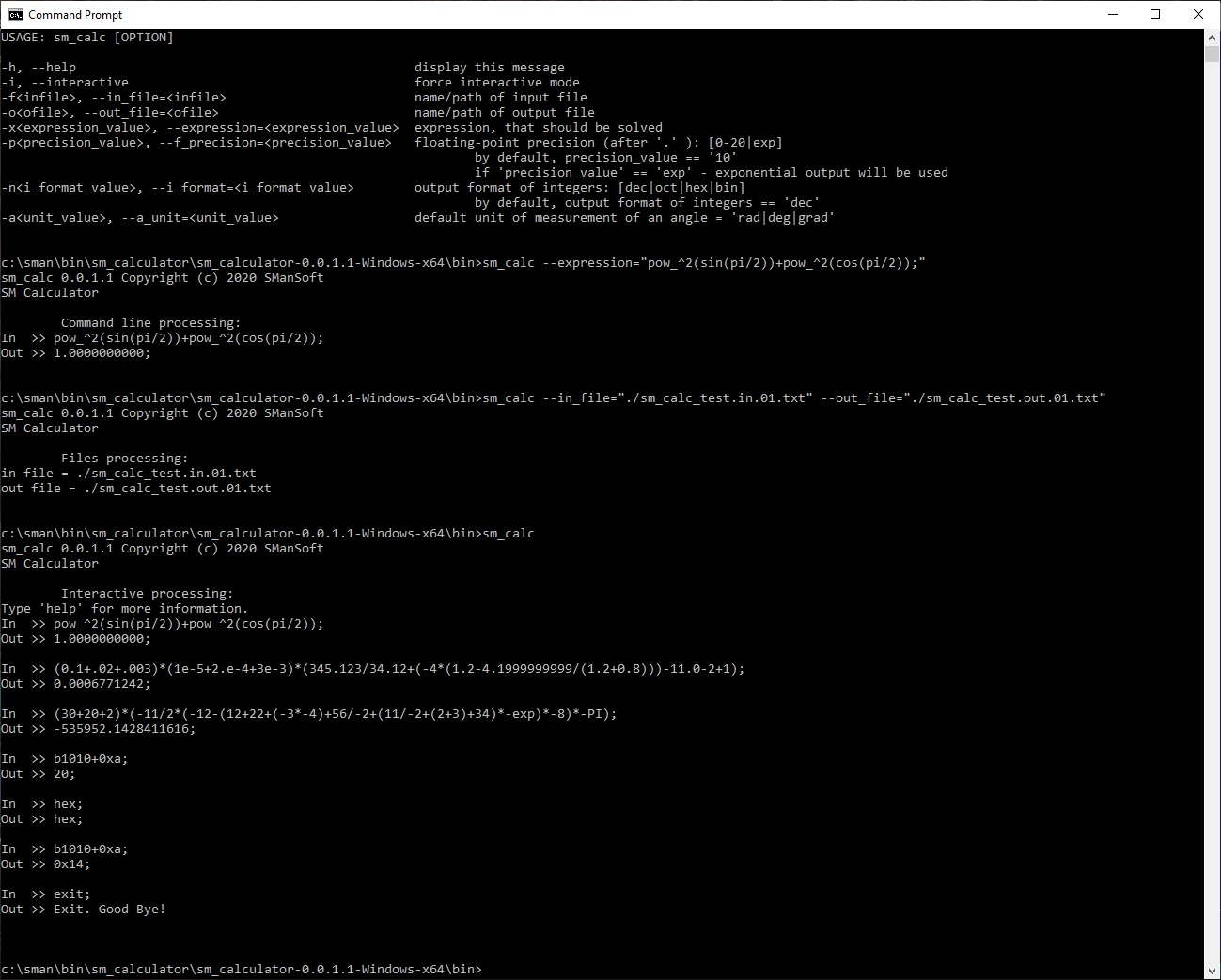Demo of the sm_calculator (SM Calculator) tool 0.0.1.1 (Linux):
—————-

Here are the results of the execution of the sm_calculator (SM Calculator) tool 0.0.1.1 on Linux Debian 10 (Buster) x64.

The sm_calculator (SM Calculator) tool 0.0.1.1 has been launched 4 times:

1. sm_calc --help
2. sm_calc --expression="pow_^2(sin(pi/2))+pow_^2(cos(pi/2));"
3. sm_calc --in_file="./sm_calc_test.in.02.txt" --out_file="./sm_calc_test.out.02.txt"
4. sm_calc

Results of execution (log file and screenshots) below:
sm_calc.log